Floating-Point Unit

The Intel Architecture Floating-Point Unit (FPU) provides high-performance floating-point processing capabilities. It supports the real, integer, and BCD-integer data types and the floating-point processing algorithms and exception handling architecture defined in the IEEE 754 and 854 Standards for Floating-Point Arithmetic. The FPU executes instructions from the processor's normal instruction stream and greatly improves the efficiency of Intel Architecture processors in handling the types of high-precision floating-point processing operations commonly found in scientific, engineering, and business applications.

This chapter describes the data types that the FPU operates on, the FPU's execution environment, and the FPU-specific instruction set. Detailed descriptions of the FPU instructions are given in "Instruction Page Key".

Compatibility and Ease of Use of the Intel ARchitecture FPU

The architecture of the Intel Architecture FPU has evolved in parallel with the architecture of early Intel Architecture processors. The first Intel Math Coprocessors (the Intel 8087, Intel 287, and Intel 387) were companion processors to the Intel 8086/8088, Intel 286, and Intel386 processors, respectively, and were designed to improve and extend the numeric processing capability of the Intel Architecture. The Intel486 DX processor for the first time integrated the CPU and the FPU architectures on one chip. The Pentium processor's FPU offered the same architecture as the Intel486 DX processor's FPU, but with improved performance. The Pentium Pro processor's FPU further extended the floating-point processing capability of Intel Architecture family of processors and added several new instructions to improve processing throughput.

Throughout this evolution, compatibility among the various generations of FPUs and math coprocessors has been maintained. For example, the Pentium Pro processor's FPU is fully compatible with the Pentium and Intel486 DX processors's FPUs.

Each generation of the Intel Architecture FPUs have been explicitly designed to deliver stable, accurate results when programmed using straightforward "pencil and paper" algorithms, bringing the functionality and power of accurate numeric computation into the hands of the general user. The IEEE 754 standard specifically addresses this issue, recognizing the fundamental importance of making numeric computations both easy and safe to use.

For example, some processors can overflow when two single-precision floating-point numbers are multiplied together and then divided by a third, even if the final result is a perfectly valid 32-bit number. The Intel Architecture FPUs deliver the correctly rounded result. Other typical examples of undesirable machine behavior in straightforward calculations occur when computing financial rate of return, which involves the expression (1 + i)n or when solving for roots of a quadratic equation:If a does not equal 0, the formula is numerically unstable when the roots are nearly coincident or when their magnitudes are wildly different. The formula is also vulnerable to spurious over/underflows when the coefficients a, b, and c are all very big or all very tiny. When single-precision (4-byte) floating-point coefficients are given as data and the formula is evaluated in the FPU's normal way, keeping all intermediate results in its stack, the FPU produces impeccable single-precision roots. This happens because, by default and with no effort on the programmer's part, the FPU evaluates all those sub-expressions with so much extra precision and range as to overwhelm almost any threat to numerical integrity.

If double-precision data and results were at issue, a better formula would have to be used, and once again the FPU's default evaluation of that formula would provide substantially enhanced numerical integrity over mere double-precision evaluation.

On most machines, straightforward algorithms will not deliver consistently correct results (and will not indicate when they are incorrect). To obtain correct results on traditional machines under all conditions usually requires sophisticated numerical techniques that go beyond typical programming practice. General application programmers using straightforward algorithms will produce much more reliable programs using the Intel architectures. This simple fact greatly reduces the software investment required to develop safe, accurate computation-based products.

Beyond traditional numeric support for scientific applications, the Intel architectures have built-in facilities for commercial computing. They can process decimal numbers of up to 18 digits without round-off errors, performing exact arithmetic on integers as large as 264 (or 1018). Exact arithmetic is vital in accounting applications where rounding errors may introduce monetary losses that cannot be reconciled.

The Intel FPU's contain a number of optional numerical facilities that can be invoked by sophisticated users. These advanced features include directed rounding, gradual underflow, and programmed exception-handling facilities.

These automatic exception-handling facilities permit a high degree of flexibility in numeric processing software, without burdening the programmer. While performing numeric calculations, the processor automatically detects exception conditions that can potentially damage a calculation (for example, X ÷ 0 or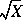when X < 0). By default, on-chip exception logic handles these exceptions so that a reasonable result is produced and execution may proceed without program interruption. Alternatively, the processor can invoke a software exception handler to provide special results whenever various types of exceptions are detected.

Real Numbers and Floating-Point Formats

This section describes how real numbers are represented in floating-point format in the Intel Architecture FPU. It also introduces terms such as normalized numbers, denormalized numbers, biased exponents, signed zeros, and NaNs. Readers who are already familiar with floating-point processing techniques and the IEEE standards may wish to skip this section.

Real Number System

As shown in Figure 31-1, the real-number system comprises the continuum of real numbers from minus infinity (-·) to plus infinity (+·).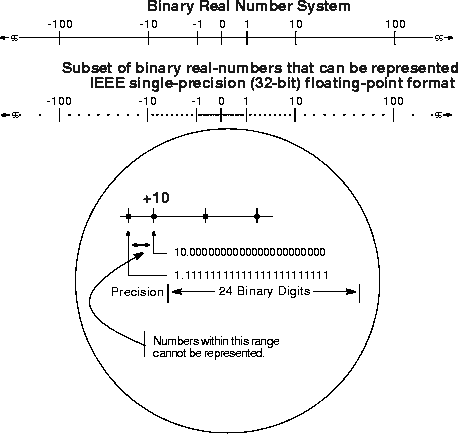Because the size and number of registers that any computer can have is limited, only a subset of the real-number continuum can be used in real-number calculations. As shown at the bottom of Figure 31-1, the subset of real numbers that a particular FPU supports represents an approximation of the real number system. The range and precision of this real-number subset is determined by the format that the FPU uses to represent real numbers.

Floating-Point Format

To increase the speed and efficiency of real-number computations, computers or FPUs typically represent real numbers in a binary floating-point format. In this format, a real number has three parts: a sign, a significand, and an exponent. Figure 31-2 shows the binary floating-point format that the Intel Architecture FPU uses. This format conforms to the IEEE standard.

The sign is a binary value that indicates whether the number is positive (0) or negative (1). The significand has two parts: a 1-bit binary integer (also referred to as the J-bit) and a binary fraction. The J-bit is often not represented, but instead is an implied value. The exponent is a binary integer that represents the base-2 power that the significand is raised to.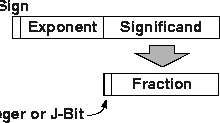Table 7-1 shows how the real number 178.125 (in ordinary decimal format) is stored in floating-point format. The table lists a progression of real number notations that leads to the single-real, 32-bit floating-point format (which is one of the floating-point formats that the FPU supports). In this format, the significand is normalized (see "Normalized Numbers") and the exponent is biased (see "Biased Exponent"). For the single-real format, the biasing constant is +127.

Normalized Numbers

In most cases, the FPU represents real numbers in normalized form. This means that except for zero, the significand is always made up of an integer of 1 and the following fraction:

• 1.fff...ff

For values less than 1, leading zeros are eliminated. (For each leading zero eliminated, the exponent is decremented by one.)

Real Number Notation
Notation
Value
Ordinary Decimal
178.125
Scientific Decimal   1.78125E102
Scientific Binary
1.0110010001E2111
Scientific Binary
(Biased Exponent)

1.0110010001E210000110

Single-Real Format
Sign   Biased Exponent   Normalized Significand
0   10000110   01100100010000000000000   1. (Implied)

Representing numbers in normalized form maximizes the number of significant digits that can be accommodated in a significand of a given width. To summarize, a normalized real number consists of a normalized significand that represents a real number between 1 and 2 and an exponent that specifies the number's binary point.

Biased Exponent

The FPU represents exponents in a biased form. This means that a constant is added to the actual exponent so that the biased exponent is always a positive number. The value of the biasing constant depends on the number of bits available for representing exponents in the floating-point format being used. The biasing constant is chosen so that the smallest normalized number can be reciprocated without overflow.

(See "Real Numbers" for a list of the biasing constants that the FPU uses for the various sizes of real data-types.)

Real Number and Non-number Encodings

A variety of real numbers and special values can be encoded in the FPU's floating-point format. These numbers and values are generally divided into the following classes:

• Signed zeros.
• Denormalized finite numbers.
• Normalized finite numbers.
• Signed infinities.
• NaNs.
• Indefinite numbers.

(The term NaN stands for "Not a Number.")

Figure 31-3 shows how the encodings for these numbers and non-numbers fit into the real number continuum. The encodings shown here are for the IEEE single-precision (32-bit) format, where the term "S" indicates the sign bit, "E" the biased exponent, and "F" the fraction. (The exponent values are given in decimal.)

The FPU can operate on and/or return any of these values, depending on the type of computation being performed. The following sections describe these number and non-number classes.

Signed Zeros

Zero can be represented as a +0 or a -0 depending on the sign bit. Both encodings are equal in value. The sign of a zero result depends on the operation being performed and the rounding mode being used. Signed zeros have been provided to aid in implementing interval arithmetic. The sign of a zero may indicate the direction from which underflow occurred, or it may indicate the sign of an · that has been reciprocated.

Normalized and Denormalized Finite Numbers

Non-zero, finite numbers are divided into two classes: normalized and denormalized. The normalized finite numbers comprise all the non-zero finite values that can be encoded in a normalized real number format between zero and ·. In the single-real format shown in Figure 31-3, this group of numbers includes all the numbers with biased exponents ranging from 1 to 25410 (unbiased, the exponent range is from -12610 to +12710).When real numbers become very close to zero, the normalized-number format can no longer be used to represent the numbers. This is because the range of the exponent is not large enough to compensate for shifting the binary point to the right to eliminate leading zeros.

When the biased exponent is zero, smaller numbers can only be represented by making the integer bit (and perhaps other leading bits) of the significand zero. The numbers in this range are called denormalized (or tiny) numbers. The use of leading zeros with denormalized numbers allows smaller numbers to be represented. However, this denormalization causes a loss of precision (the number of significant bits in the fraction is reduced by the leading zeros).

When performing normalized floating-point computations, an FPU normally operates on normalized numbers and produces normalized numbers as results. Denormalized numbers represent an underflow condition.

A denormalized number is computed through a technique called gradual underflow. Table 31-2 gives an example of gradual underflow in the denormalization process. Here the single-real format is being used, so the minimum exponent (unbiased) is -12610. The true result in this example requires an exponent of -12910 in order to have a normalized number. Since -12910 is beyond the allowable exponent range, the result is denormalized by inserting leading zeros until the minimum exponent of -12610 is reached.

Denormalization Process
Operation   Sign   Exponent*   Significand
True Result   0   -129   1.01011100000...00
Denormalize   0   -128   0.10101110000...00
Denormalize   0   -127   0.01010111000...00
Denormalize   0   -126   0.00101011100...00
Denormal Result   0   -126   0.00101011100...00
NOTE: * Expressed as an unbiased, decimal number.

In the extreme case, all the significant bits are shifted out to the right by leading zeros, creating a zero result.

The FPU deals with denormal values in the following ways:

• It avoids creating denormals by normalizing numbers whenever possible.
• It provides the floating-point underflow exception to permit programmers to detect cases when denormals are created.
• It provides the floating-point denormal-operand exception to permit procedures or programs to detect when denormals are being used as source operands for computations.

When a denormal number in single- or double-real format is used as a source operand and the denormal exception is masked, the FPU automatically normalizes the number when it is converted to extended-real format.

Signed Infinities

The two infinities, +· and -·, represent the maximum positive and negative real numbers, respectively, that can be represented in the floating-point format. Infinity is always represented by a zero significand (fraction and integer bit) and the maximum biased exponent allowed in the specified format (for example, 25510 for the single-real format).

The signs of infinities are observed, and comparisons are possible. Infinities are always interpreted in the affine sense; that is, -· is less than any finite number and +· is greater than any finite number. Arithmetic on infinities is always exact. Exceptions are generated only when the use of an infinity as a source operand constitutes an invalid operation.

Whereas denormalized numbers represent an underflow condition, the two infinity numbers represent the result of an overflow condition. Here, the normalized result of a computation has a biased exponent greater than the largest allowable exponent for the selected result format.

NaNs

Since NaNs are non-numbers, they are not part of the real number line. In Figure 31-3, the encoding space for NaNs in the FPU floating-point formats is shown above the ends of the real number line. This space includes any value with the maximum allowable biased exponent and a non-zero fraction. (The sign bit is ignored for NaNs.)

The IEEE standard defines two classes of NaN: quiet NaNs (QNaNs) and signaling NaNs (SNaNs). A QNaN is a NaN with the most significant fraction bit set; an SNaN is a NaN with the most significant fraction bit clear. QNaNs are allowed to propagate through most arithmetic operations without signaling an exception. SNaNs generally signal an invalid-operation exception whenever they appear as operands in arithmetic operations. Exceptions are discussed in "Floating-Point Exception Handling".

See "Operating on NaNs", for detailed information on how the FPU handles NaNs.

Indefinite

For each FPU data type, one unique encoding is reserved for representing the special value indefinite. For example, when operating on real values, the real indefinite value is a QNaN (see "Real Numbers"). The FPU produces indefinite values as responses to masked floating-point exceptions.

FPU Architecture

From an abstract, architectural view, the FPU is a coprocessor that operates in parallel with the processor's integer unit (see Figure 31-4). The FPU gets its instructions from the same instruction decoder and sequencer as the integer unit and shares the system bus with the integer unit. Other than these connections, the integer unit and FPU operate independently and in parallel. (The actual microarchitecture of an Intel Architecture processor varies among the various families of processors. For example, the Pentium Pro processor has two integer units and two FPUs; whereas, the Pentium processor has two integer units and one FPU, and the Intel486 processor has one integer unit and one FPU.)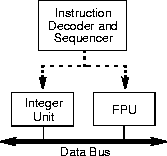The instruction execution environment of the FPU (see Figure 31-5) consists of 8 data registers (called the FPU data registers) and the following special-purpose registers:

• The status register.
• The control register.
• The tag word register.
• Instruction pointer register.
• Last operand (data pointer) register.
• Opcode register.

These registers are described in the following sections.

The FPU Data Registers

The FPU data registers (shown in Figure 31-5) consist of eight 80-bit registers. Values are stored in these registers in the extended-real format shown in Figure 31-17. When real, integer, or packed BCD integer values (in any of the formats shown in Figure 31-17) are loaded from memory into any of the FPU data registers, the values are automatically converted into extended-real format (if they are not already in that format). When computation results are subsequently transferred back into memory from any of the FPU registers, the results can be left in the extended-real format or converted back into one of the other FPU formats (real, integer, or packed BCD integers) shown in Figure 31-17.

The FPU instructions treat the eight FPU data registers as a register stack (see Figure 31-6). All addressing of the data registers is relative to the register on the top of the stack. The register number of the current top-of-stack register is stored in the TOP (stack TOP) field in the FPU status word. Load operations decrement TOP by one and load a value into the new top-of-stack register, and store operations store the value from the current TOP register in memory and then increment TOP by one. (For the FPU, a load operation is equivalent to a push and a store operation is equivalent to a pop.)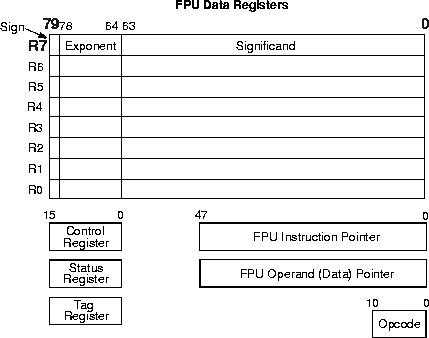If a load operation is performed when TOP is at 0, register wraparound occurs and the new value of TOP is set to 7. The floating-point stack-overflow exception indicates when wraparound might cause an unsaved value to be overwritten (see "Stack Overflow or Underflow Exception (#IS)").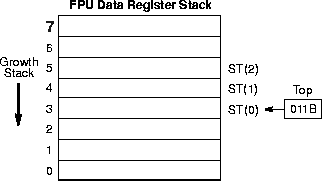Many floating-point instructions have several addressing modes that permit the programmer to implicitly operate on the top of the stack, or to explicitly operate on specific registers relative to the TOP. Assemblers supports these register addressing modes, using the expression ST(0), or simply ST, to represent the current stack top and ST(i) to specify the ith register from TOP in the stack (0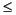i7). For example, if TOP contains 011B (register 3 is the top of the stack), the following instruction would add the contents of two registers in the stack (registers 3 and 5):

```FADD ST, ST(2);```

Figure 31-7 shows an example of how the stack structure of the FPU registers and instructions are typically used to perform a series of computations. Here, a two-dimensional dot product is computed, as follows:

1. The first instruction (FLD value1) decrements the stack register pointer (TOP) and loads the value 5.6 from memory into ST(0). The result of this operation is shown in snap-shot (a).

2. The second instruction multiplies the value in ST(0) by the value 2.4 from memory and stores the result in ST(0), shown in snap-shot (b).

3. The third instruction decrements TOP and loads the value 3.8 in ST(0).

4. The fourth instruction multiplies the value in ST(0) by the value 10.3 from memory and stores the result in ST(0), shown in snap-shot (c).

5. The fifth instruction adds the value and the value in ST(1) and stores the result in ST(0), shown in snap-shot (d).

The style of programming demonstrated in this example is supported by the floating-point instruction set. In cases where the stack structure causes computation bottlenecks, the FXCH (exchange FPU register contents) instruction can be used to streamline a computation.

Parameter Passing With the FPU Register Stack

Like the general-purpose registers in the processor's integer unit, the contents of the FPU data registers are unaffected by procedure calls, or in other words, the values are maintained across procedure boundaries. A calling procedure can thus use the FPU data registers (as well as the procedure stack) for passing parameter between procedures. The called procedure can reference parameters passed through the register stack using the current stack register pointer (TOP) and the ST(0) and ST(i) nomenclature. It is also common practice for a called procedure to leave a return value or result in register ST(0) when returning execution to the calling procedure or program.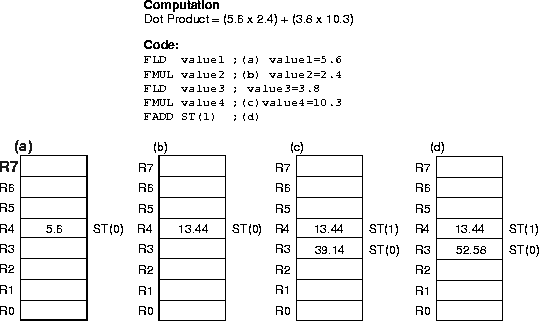FPU Status Register

The 16-bit FPU status register (see in Figure 31-8) indicates the current state of the FPU. The flags in the FPU status register include the FPU busy flag, top-of-stack (TOP) pointer, condition code flags, error summary status flag, stack fault flag, and exception flags. The FPU sets the flags in this register to show the results of operations.

The contents of the FPU status register (referred to as the FPU status word) can be stored in memory using the FSTSW/FNSTSW, FSTENV/FNSTENV, and FSAVE/FNSAVE instructions. It can also be stored in the AX register of the integer unit, using the FSTSW/FNSTSW instructions.

Top of Stack (TOP) Pointer

A pointer to the FPU data register that is currently at the top of the FPU register stack is contained in bits 11 through 13 of the FPU status word. This pointer, which is commonly referred to as TOP (for top-of-stack), is a binary value from 0 to 7. See "The FPU Data Registers", for more information about the TOP pointer.

Condition Code Flags

The four FPU condition code flags (C0 through C3) indicate the results of floating-point comparison and arithmetic operations. Table 31-3 summarizes the manner in which the floating-point instructions set the condition code flags. These condition code bits are used principally for conditional branching and for storage of information used in exception handling (see "Branching and Conditional Moves on FPU Condition Codes").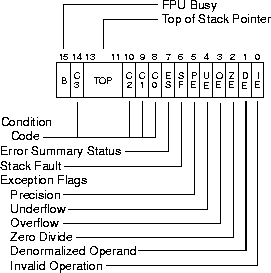As shown in Table 31-3, the C1 condition code flag is used for a variety of functions. When both the IE and SF flags in the FPU status word are set, indicating a stack overflow or underflow exception (#IS), the C1 flag distinguishes between overflow (C1=1) and underflow (C1=0). When the PE flag in the status word is set, indicating an inexact (rounded) result, the C1 flag is set to 1 if the last rounding by the instruction was upward. The FXAM instruction sets C1 to the sign of the value being examined.

The C2 condition code flag is used by the FPREM and FPREM1 instructions to indicate an incomplete reduction (or partial remainder). When a successful reduction has been completed, the C0, C3, and C1 condition code flags are set to the three least-significant bits of the quotient (Q2, Q1, and Q0, respectively). See "FPREM1-Partial Remainder" in Chapter 3, Instruction Set Reference, of the Intel Architecture Software Developer's Manual, Volume 2, for more information on how these instructions use the condition code flags.

The FPTAN, FSIN, FCOS, and FSINCOS instructions set the C2 flag to 1 to indicate that the source operand is beyond the allowable range of ±263.

Where the state of the condition code flags are listed as undefined in Table 31-3, do not rely on any specific value in these flags.

FPU Condition Code Interpretation
Instruction   C0   C3   C2   C1
FCOM, FCOMP, FCOMPP, FICOM, FICOMP, FTST, FUCOM, FUCOMP, FUCOMPP
Result of Comparison
Operands
are not Comparable
0 or #IS
FCOMI, FCOMIP, FUCOMI, FUCOMIP
Undefined. (These instructions set the
status flags in the EFLAGS register.)
#IS
FXAM
Operand class
Sign
FPREM, FPREM1   Q2   Q1   0=reduction complete   1=reduction incomplete   Q0 or #IS
F2XM1, FADD, FADDP, FBSTP, FCMOVcc, FIADD, FDIV, FDIVP, FDIVR, FDIVRP, FIDIV, FIDIVR, FIMUL, FIST, FISTP, FISUB, FISUBR,FMUL, FMULP, FPATAN, FRNDINT, FSCALE, FST, FSTP, FSUB, FSUBP, FSUBR, FSUBRP,FSQRT, FYL2X, FYL2XP1
Undefined
Roundup or #IS
FCOS, FSIN, FSINCOS, FPTAN
Undefined
1=source operand out of range.   Roundup or #IS (Undefined if C2=1)
FABS, FBLD, FCHS, FDECSTP, FILD, FINCSTP, FLD, Load Constants, FSTP (ext. real), FXCH, FXTRACT
Undefined
0 or #IS
FLDENV, FRSTOR
FFREE, FLDCW, FCLEX/FNCLEX, FNOP, FSTCW/FNSTCW, FSTENV/FNSTENV, FSTSW/FNSTSW,
Undefined
FINIT/FNINIT, FSAVE/FNSAVE   0   0   0   0

Exception Flags

The six exception flags (bits 0 through 5) of the status word indicate that one or more floating-point exceptions has been detected since the bits were last cleared. The individual exception flags (IE, DE, ZE, OE, UE, and PE) are described in detail in "Floating-Point Exception Handling". Each of the exception flags can be masked by an exception mask bit in the FPU control word (see "FPU Control Word"). The exception summary status (ES) flag (bit 7) is set when any of the unmasked exception flags are set. When the ES flag is set, the FPU exception handler is invoked, using one of the techniques described in "Software Exception Handling". (Note that if an exception flag is masked, the FPU will still set the flag if its associated exception occurs, but it will not set the ES flag.)

The exception flags are "sticky" bits, meaning that once set, they remain set until explicitly cleared. They can be cleared by executing the FCLEX/FNCLEX (clear exceptions) instructions, by reinitializing the FPU with the FINIT/FNINIT or FSAVE/FNSAVE instructions, or by overwriting the flags with an FRSTOR or FLDENV instruction.

The B-bit (bit 15) is included for 8087 compatibility only. It reflects the contents of the ES flag.

Stack Fault Flag

The stack fault flag (bit 6 of the FPU status word) indicates that stack overflow or stack underflow has occurred. The FPU explicitly sets the SF flag when it detects a stack overflow or underflow condition, but it does not explicitly clear the flag when it detects an invalid-arithmetic-operand condition. When this flag is set, the condition code flag C1 indicates the nature of the fault: overflow (C1 = 1) and underflow (C1 = 0). The SF flag is a "sticky" flag, meaning that after it is set, the processor does not clear it until it is explicitly instructed to do so (for example, by an FINIT/FNINIT, FCLEX/FNCLEX, or FSAVE/FNSAVE instruction).

Branching and Conditional Moves on FPU Condition Codes

The Intel Architecture FPU (beginning with the Pentium Pro processor) supports two mechanisms for branching and performing conditional moves according to comparisons of two floating-point values. These mechanism are referred to here as the "old mechanism" and the "new mechanism."

The old mechanism is available in FPU's prior to the Pentium Pro processor and in the Pentium Pro processor. This mechanism uses the floating-point compare instructions (FCOM, FCOMP, FCOMPP, FTST, FUCOMPP, FICOM, and FICOMP) to compare two floating-point values and set the condition code flags (C0 through C3) according to the results. The contents of the condition code flags are then copied into the status flags of the EFLAGS register using a two step process (see Figure 31-9):

1. The FSTSW AX instruction moves the FPU status word into the AX register.

2. The SAHF instruction copies the upper 8 bits of the AX register, which includes the condition code flags, into the lower 8 bits of the EFLAGS register.

When the condition code flags have been loaded into the EFLAGS register, conditional jumps or conditional moves can be performed based on the new settings of the status flags in the EFLAGS register.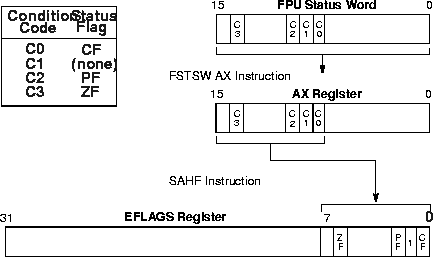The new mechanism is available only in the Pentium Pro processor. Using this mechanism, the new floating-point compare and set EFLAGS instructions (FCOMI, FCOMIP, FUCOMI, and FUCOMIP) compare two floating-point values and set the ZF, PF, and CF flags in the EFLAGS register directly. A single instruction thus replaces the three instructions required by the old mechanism.

Note also that the FCMOVcc instructions (also new in the Pentium Pro processor) allow conditional moves of floating-point values (values in the FPU data registers) based on the setting of the status flags (ZF, PF, and CF) in the EFLAGS register. These instructions eliminate the need for an IF statement to perform conditional moves of floating-point values.

FPU Control Word

The 16-bit FPU control word (see in Figure 31-10) controls the precision of the FPU and rounding method used. It also contains the exception-flag mask bits. The control word is cached in the FPU control register. The contents of this register can be loaded with the FLDCW instruction and stored in memory with the FSTCW/FNSTCW instructions.

When the FPU is initialized with either an FINIT/FNINIT or FSAVE/FNSAVE instruction, the FPU control word is set to 037FH, which masks all floating-point exceptions, sets rounding to nearest, and sets the FPU precision to 64 bits.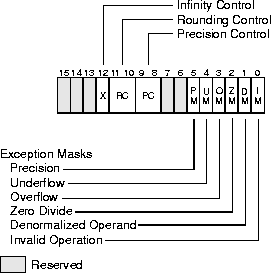The exception-flag mask bits (bits 0 through 5 of the FPU control word) mask the 6 exception flags in the FPU status word (also bits 0 through 5). When one of these mask bits is set, its corresponding floating-point exception is blocked from being generated.

Precision Control Field

The precision-control (PC) field (bits 8 and 9 of the FPU control word) determines the precision (64, 53, or 24 bits) of floating-point calculations made by the FPU (see Table 31-4). The default precision is extended precision, which uses the full 64-bit significand available with the extended-real format of the FPU data registers. This setting is best suited for most applications, because it allows applications to take full advantage of the precision of the extended-real format.

Precision Control Field (PC)
Precision   PC Field
Single Precision (24-Bits*)   00B
Reserved   01B
Double Precision (53-Bits*)   10B
Extended Precision (64-Bits)   11B
NOTE: *Includes the implied integer bit.

The double precision and single precision settings, reduce the size of the significand to 53 bits and 24 bits, respectively. These settings are provided to support the IEEE standard and to allow exact replication of calculations which were done using the lower precision data types. Using these settings nullifies the advantages of the extended-real format's 64-bit significand length. When reduced precision is specified, the rounding of the significand value clears the unused bits on the right to zeros.

The precision-control bits only affect the results of the following floating-point instructions: FADD, FADDP, FSUB, FSUBP, FSUBR, FSUBRP, FMUL, FMULP, FDIV, FDIVP, FDIVR, FDIVRP, and FSQRT.

Rounding Control Field

The rounding-control (RC) field of the FPU control register (bits 10 and 11) controls how the results of floating-point instructions are rounded. Four rounding modes are supported (see Table 31-5): round to nearest, round up, round down, and round toward zero. Round to nearest is the default rounding mode and is suitable for most applications. It provides the most accurate and statistically unbiased estimate of the true result.

Rounding Control Field (RC)
Rounding Mode   RC Field
Setting

Description

Round to nearest (even)   00B   Rounded result is the closest to the infinitely precise result. If two values are equally close, the result is the even value (that is, the one with the least-significant bit of zero).
Round down (toward -·)   01B   Rounded result is close to but no greater than the infinitely precise result.
Round up (toward +·)   10B   Rounded result is close to but no less than the infinitely precise result.
Round toward zero (Truncate)   11B   Rounded result is close to but no greater in absolute value than the infinitely precise result.

The round up and round down modes are termed directed rounding and can be used to implement interval arithmetic. Interval arithmetic is used to determine upper and lower bounds for the true result of a multistep computation, when the intermediate results of the computation are subject to rounding.

The round toward zero mode (sometimes called the "chop" mode) is commonly used when performing integer arithmetic with the FPU.

Whenever possible, the FPU produces an infinitely precise result in the destination format (single, double, or extended real). However, it is often the case that the infinitely precise result of an arithmetic or store operation cannot be encoded exactly in the format of the destination operand.

For example, the following value (a) has a 24-bit fraction. The least-significant bit of this fraction (the underlined bit) cannot be encoded exactly in the single-real format (which has only a 23-bit fraction):

(a) 1.0001 0000 1000 0011 1001 0111E2 101

To round this result (a), the FPU first selects two representable fractions b and c that most closely bracket a in value (b < a < c).

(b) 1.0001 0000 1000 0011 1001 011E2 101

(c) 1.0001 0000 1000 0011 1001 100E2 101

The FPU then sets the result to b or to c according to the rounding mode selected in the RC field. Rounding introduces an error in a result that is less than one unit in the last place to which the result is rounded.

The rounded result is called the inexact result. When the FPU produces an inexact result, the floating-point precision (inexact) flag (PE) is set in the FPU status word.

When the overflow exception is masked and the infinitely precise result is between the largest positive finite value allowed in a particular format and +·, the FPU rounds the result as shown in Table 31-6.

 Rounding Mode Result Rounding to nearest (even) +· Rounding toward zero (Truncate) Maximum, positive finite value Rounding up (toward +·) +· Rounding down) (toward -·) Maximum, positive finite value

When the overflow exception is masked and the infinitely precise result is between the largest negative finite value allowed in a particular format and -·, the FPU rounds the result as shown in Table 31-7.

 Rounding Mode Result Rounding to nearest (even) -· Rounding toward zero (Truncate) Maximum, negative finite value Rounding up (toward +·) Maximum, negative finite value Rounding down) (toward -·) -·

The rounding modes have no effect on comparison operations, operations that produce exact results, or operations that produce NaN results.

Infinity Control Flag

The infinity control flag (bit 12 of the FPU control word) is provided for compatibility with the Intel 287 Math Coprocessor; it is not meaningful for the Pentium Pro processor FPU or for the Pentium processor FPU, the Intel486 processor FPU, or Intel 387 processor NPX. See "Signed Infinities", for information on how the Intel Architecture FPUs handle infinity values.

FPU Tag Word

The 16-bit tag word (see in Figure 31-11) indicates the contents of each the 8 registers in the FPU data-register stack (one 2-bit tag per register). The tag codes indicate whether a register contains a valid number, zero, or a special floating-point number (NaN, infinity, denormal, or unsupported format), or whether it is empty. The FPU tag word is cached in the FPU in the FPU tag word register. When the FPU is initialized with either an FINIT/FNINIT or FSAVE/FNSAVE instruction, the FPU tag word is set to FFFFH, which marks all the FPU data registers as empty.

.Each tag in the FPU tag word corresponds to a physical register (numbers 0 through 7). The current top-of-stack (TOP) pointer stored in the FPU status word can be used to associate tags with registers relative to ST(0).

The FPU uses the tag values to detect stack overflow and underflow conditions. Stack overflow occurs when the TOP pointer is decremented (due to a register load or push operation) to point to a non-empty register. Stack underflow occurs when the TOP pointer is incremented (due to a save or pop operation) to point to an empty register or when an empty register is also referenced as a source operand. A non-empty register is defined as a register containing a zero (01), a valid value (00), or an special (10) value.

Application programs and exception handlers can use this tag information to check the contents of an FPU data register without performing complex decoding of the actual data in the register. To read the tag register, it must be stored in memory using either the FSTENV/FNSTENV or FSAVE/FNSAVE instructions. The location of the tag word in memory after being saved with one of these instructions is shown in Figure 31-13 through 31-16.

Software cannot directly load or modify the tags in the tag register. The FLDENV and FRSTOR instructions load an image of the tag register into the FPU; however, the FPU uses those tag values only to determine if the data registers are empty (11B) or non-empty (00B, 01B, or 10B). If the tag register image indicates that a data register is empty, the tag in the tag register for that data register is marked empty (11B); if the tag register image indicates that the data register is non-empty, the FPU reads the actual value in the data register and sets the tag for the register accordingly. This action prevents a program from setting the values in the tag register to incorrectly represent the actual contents of non-empty data registers.

The FPU Instruction and Operand (Data) Pointers

The FPU stores pointers to the instruction and operand (data) for the last non-control instruction executed in two 48-bit registers: the FPU instruction pointer and FPU operand (data) pointer registers (see Figure 31-5). (This information is saved to provide state information for exception handlers.)

The contents of the FPU instruction and operand pointer registers remain unchanged when any of the control instructions (FINIT/FNINIT, FCLEX/FNCLEX, FLDCW, FSTCW/FNSTCW, FSTSW/FNSTSW, FSTENV/FNSTENV, FLDENV, FSAVE/FNSAVE, FRSTOR, and WAIT/FWAIT) are executed. The contents of the FPU operand register are undefined if the prior non-control instruction did not have a memory operand.

The pointers stored in the FPU instruction and operand pointer registers consist of an offset (stored in bits 0 through 31) and a segment selector (stored in bits 32 through 47).

These registers can be accessed by the FSTENV/FNSTENV, FLDENV, FINIT/FNINIT, FSAVE/FNSAVE and FRSTOR instructions. The FINIT/FNINIT and FSAVE/FNSAVE instructions clear these registers.

For all the Intel Architecture FPUs and NPXs except the 8087, the FPU instruction pointer points to any prefixes that preceded the instruction. For the 8087, the FPU instruction pointer points only to the actual opcode.

Last Instruction Opcode

The FPU stores the opcode of the last non-control instruction executed in an 11-bit FPU opcode register. (This information provides state information for exception handlers.) Only the first and second opcode bytes (after all prefixes) are stored in the FPU opcode register. Figure 31-12 shows the encoding of these two bytes. Since the upper 5 bits of the first opcode byte are the same for all floating-point opcodes (11011B), only the lower 3 bits of this byte are stored in the opcode register.

Saving the FPU's State

The FSTENV/FNSTENV and FSAVE/FNSAVE instructions store FPU state information in memory for use by exception handlers and other system and application software. The FSTENV/FNSTENV instruction saves the contents of the status, control, tag, FPU instruction pointer, FPU operand pointer, and opcode registers. The FSAVE/FNSAVE instruction stores that information plus the contents of the FPU data registers. Note that the FSAVE/FNSAVE instruction also initializes the FPU to default values (just as the FINIT/FNINIT instruction does) after it has saved the original state of the FPU.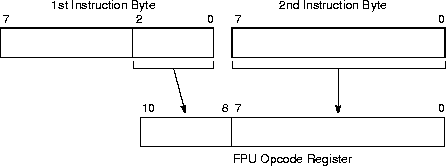The manner in which this information is stored in memory depends on the operating mode of the processor (protected mode or real-address mode) and on the operand-size attribute in effect (32-bit or 16-bit). See Figure 31-13 through 31-16. In virtual-8086 mode or SMM, the real-address mode formats shown in Figure 31-16 is used. See "Using the FPU in SMM" in Chapter 11 of the Intel Architecture Software Developer's Manual, Volume 3, for special considerations for using the FPU while in SMM.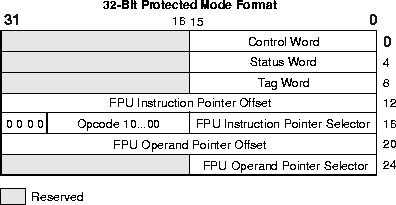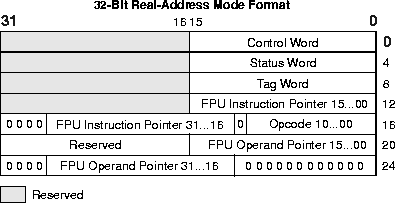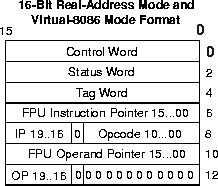The FLDENV and FRSTOR instructions allow FPU state information to be loaded from memory into the FPU. Here, the FLDENV instruction loads only the status, control, tag, FPU instruction pointer, FPU operand pointer, and opcode registers, and the FRSTOR instruction loads all the FPU registers, including the FPU stack registers.

Floating-Point Data Types and Formats

The Intel Architecture FPU recognizes and operates on seven data types, divided into three groups: reals, integers, and packed BCD integers. Figure 31-17 shows the data formats for each of the FPU data types. Table 31-8 gives the length, precision, and approximate normalized range that can be represented of each FPU data type. Denormal values are also supported in each of the real types, as required by IEEE Std. 854.

With the exception of the 80-bit extended-real format, all of these data types exist in memory only. When they are loaded into FPU data registers, they are converted into extended-real format and operated on in that format.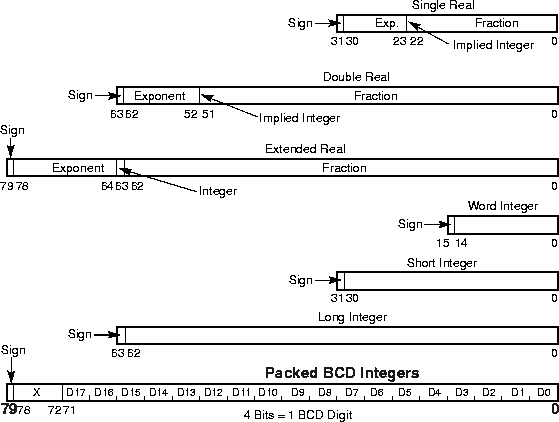When stored in memory, the least significant byte of an FPU data-type value is stored at the initial address specified for the value. Successive bytes from the value are then stored in successively higher addresses in memory. The floating-point instructions load and store memory operands using only the initial address of the operand.

Real Numbers

The FPU's three real data types (single-real, double-real, and extended-real) correspond directly to the single-precision, double-precision, and double-extended-precision formats in the IEEE standard. The extended-precision format is the format used by the data registers in the FPU. Table 31-8 gives the precision and range of these data types and Figure 31-17 gives the formats.

For the single-real and double-real formats, only the fraction part of the significand is encoded. The integer is assumed to be 1 for all numbers except 0 and denormalized finite numbers. For the extended-real format, the integer is contained in bit 63, and the most-significant fraction bit is bit 62. Here, the integer is explicitly set to 1 for normalized numbers, infinities, and NaNs, and to 0 for zero and denormalized numbers.

 Data Type Length Precision(Bits) Approximate Normalized Range Binary Decimal Binary Real   Single real 32 24 2-126 to 2127 1.18 ¥ 10-38 to 3.40 ¥ 1038 Double real 64 53 2-1022 to 21023 2.23 ¥ 10-308 to 1.79 ¥ 10308 Extended real 80 64 2-16382 to 216383 3.37 ¥ 10-4932 to 1.18 ¥ 104932 Binary Integer   Word integer 16 15 -215 to 215 - 1 -32,768 to 32,767 Short integer 32 31 -231 to 231 - 1 -2.14 ¥ 109 to 2.14 ¥ 109 Long integer 64 63 -263 to 263 - 1 -9.22 ¥ 1018 to 9.22 ¥ 1018 Packed BCD Integers 80 18 (decimaldigits) Not Pertinent (-1018 + 1) to (1018 - 1)

The exponent of each real data type is encoded in biased format. The biasing constant is 127 for the single-real format, 1023 for the double-real format, and 16,383 for the extended-real format.

Table 31-9 shows the encodings for all the classes of real numbers (that is, zero, denormalized-finite, normalized-finite, and ·) and NaNs for each of the three real data-types. It also gives the format for the real indefinite value.

When storing real values in memory, single-real values are stored in 4 consecutive bytes in memory; double-real values are stored in 8 consecutive bytes; and extended-real values are stored in 10 consecutive bytes.

As a general rule, values should be stored in memory in double-real format. This format provides sufficient range and precision to return correct results with a minimum of programmer attention. The single-real format is appropriate for applications that are constrained by memory; however, it provides less precision and a greater chance of overflow. The single-real format is also useful for debugging algorithms, because rounding problems will manifest themselves more quickly in this format. The extended-real format is normally reserved for holding intermediate results in the FPU registers and constants. Its extra length is designed to shield final results from the effects of rounding and overflow/underflow in intermediate calculations. However, when an application requires the maximum range and precision of the FPU (for data storage, computations, and results), values can be stored in memory in extended-real format.

The real indefinite value is a QNaN encoding that is stored by several floating-point instructions in response to a masked floating-point invalid-operation exception (see Table 31-20).

 Class Sign Biased Exponent Significand Integer1 Fraction Positive +· 0 11..11 1 00..00 +Normals 0..0 11..10..00..01 1..1 11..11..00..00 +Denormals 0..0 00..00..00..00 0..0 11.11..00..01 +Zero 0 00..00 0 00..00 Negative -Zero 1 00..00 0 00..00 -Denormals 1..1 00..00..00..00 0..0 00..01..11..11 -Normals 1..1 00..01..11..10 1..1 00..00..11..11 -· 1 11..11 1 00..00 NaNs SNaN X 11..11 1 0X..XX2 QNaN X 11..11 1 1X..XX Real Indefinite (QNaN) 1 11..11 1 10..00 Single-Real:Double-Real:Extended-Real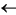8 Bits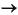11 Bits15 Bits23 Bits52 Bits63 BitsNOTE: Notes:

Integer bit is implied and not stored for single-real and double-real formats.

The fraction for SNaN encodings must be non-zero.

Binary Integers

The FPU's three binary integer data types (word, short, and long) have identical formats, except for length. Table 31-8 gives the precision and range of these data types and Figure 31-17 gives the formats. Table 31-10 gives the encodings of the three binary integer types.

 Class Sign Magnitude Positive Largest 0 11..11 . . . . . . Smallest 0 00..01 Zero 0 00..00 Negative Smallest 1 11..11 . . . . . . . . Largest 1 00..00 Integer Indefinite 1 00..00 Word Integer:Short Integer:Long Integer:15 bits31 Bits63 BitsThe most significant bit of each format is the sign bit (0 for positive and 1 for negative). Negative values are represented in standard two's complement notation. The quantity zero is represented with all bits (including the sign bit) set to zero. Note that the FPU's word-integer data type is identical to the word-integer data type used by the processor's integer unit and the short-integer format is identical to the integer unit's doubleword-integer data type.

Word-integer values are stored in 2 consecutive bytes in memory; short-integer values are stored in 4 consecutive bytes; and long-integer values are stored in 8 consecutive bytes. When loaded into the FPU's data registers, all the binary integers are exactly representable in the extended-real format.

The binary integer encoding 100..00B represents either of two things, depending on the circumstances of its use:

• The largest negative number supported by the format (-215, -231, or -263).
• The integer indefinite value.

If this encoding is used as a source operand (as in an integer load or integer arithmetic instruction), the FPU interprets it as the largest negative number representable in the format being used. If the FPU detects an invalid operation when storing an integer value in memory with an FIST/FISTP instruction and the invalid-operation exception is masked, the FPU stores the integer indefinite encoding in the destination operand as a masked response to the exception. In situations where the origin of a value with this encoding may be ambiguous, the invalid-operation exception flag can be examined to see if the value was produced as a response to an exception.

If the integer indefinite is stored in memory and is later loaded back into an FPU data register, it is interpreted as the largest negative number supported by the format.

Decimal Integers

Decimal integers are stored in a 10-byte, packed BCD format. Table 31-8 gives the precision and range of this data type and Figure 31-17 shows the format. In this format, the first 9 bytes hold 18 BCD digits, 2 digits per byte (see "BCD Integers"). The least-significant digit is contained in the lower half-byte of byte 0 and the most-significant digit is contained in the upper half-byte of byte 9. The most significant bit of byte 10 contains the sign bit (0 = positive and 1 = negative). (Bits 0 through 6 of byte 10 are don't care bits.) Negative decimal integers are not stored in two's complement form; they are distinguished from positive decimal integers only by the sign bit.

Table 31-11 gives the possible encodings of value in the decimal integer data type.

The decimal integer format exists in memory only. When a decimal integer is loaded in a data register in the FPU, it is automatically converted to the extended-real format. All decimal integers are exactly representable in extended-real format.

The packed decimal indefinite encoding is stored by the FBSTP instruction in response to a masked floating-point invalid-operation exception. Attempting to load this value with the FBLD instruction produces an undefined result.

Unsupported Extended-Real Encodings

The extended-real format permits many encodings that do not fall into any of the categories shown in Table 31-9. Table 31-12 shows these unsupported encodings. Some of these encodings were supported by the Intel 287 math coprocessor; however, most of them are not supported by the Intel 387 math coprocessor, or the internal FPUs in the Intel486, Pentium, or Pentium Pro processors. These encodings are no longer supported due to changes made in the final version of IEEE Std. 754 that eliminated these encodings.

The categories of encodings formerly known as pseudo-NaNs, pseudo-infinities, and un-normal numbers are not supported. The Intel 387 math coprocessor and the internal FPUs in the Intel486, Pentium, and Pentium Pro processors generate the invalid-operation exception when they are encountered as operands.

The encodings formerly known as pseudo-denormal numbers are not generated by the Intel 387 math coprocessor and the internal FPUs in the Intel486, Pentium, and Pentium Pro processors; however, they are used correctly when encountered as operands. The exponent is treated as if it were 00..01B and the mantissa is unchanged. The denormal exception is generated.

Packed Decimal Integer Encodings (Sheet 1 of 2)

Class

Sign

Magnitude
digit   digit   digit   digit   ...   digit
Positive   Largest     0     0000000     1001     1001     1001     1001     ...     1001
.   .       .
.   .       .
Smallest   0   0000000   0000   0000   0000   0000   ...   0001
Zero   0   0000000   0000   0000   0000   0000   ...   0000
Negative   Zero     1     0000000     0000     0000     0000     0000     ...     0000
Smallest   1   0000000   0000   0000   0000   0000   ...   0001
.   .       .
.   .       .
Largest   1   0000000   1001   1001   1001   1001   ...   1001
Decimal Integer Indefinite   1   1111111   1111   1111   UUUU*   UUUU   ...   UUUU1 byte9 bytesNOTE: * UUUU means bit values are undefined and may contain any value.

FPU Instruction Set

The floating-point instructions that the Intel Architecture FPU supports can be grouped into six functional categories:

• Data transfer instructions
• Basic arithmetic instructions
• Comparison instructions
• Transcendental instructions
• FPU control instructions

The following section briefly describes the instructions in each category. Detailed descriptions of the floating-point instructions are given in "Instruction Page Key".

Unsupported Extended-Real Encodings (Sheet 1 of 2)

Class

Sign

Biased Exponent

Significand
Integer   Fraction

Positive
Pseudo-NaNs
Quiet   0
.
0
11..11
.
11..11
0   11..11
.
10..00
Signaling   0
.
0
11..11
.
11..11
0   01..11
.
00..01

Positive Reals
Pseudo-infinity   0   11..11   0   00..00
Unnormals   0
.
0
11..10
.
00..01
0   11..11
.
00..00
Pseudo-denormals   0
.
0
00..00
.
00..00
1   11..11
.
00..00

Negative Reals
Pseudo-denormals   1
.
1
00..00
.
00..00
1   11..11
.
00..00
Unnormals   1
.
1
11..10
.
00..01
0   11..01
.
00..00
Pseudo-infinity   1   11..11   0   00..00
Negative Pseudo-NaNs     Signaling   1
.
1
11..11
.
11..11
0   01..11
.
00..01
Quiet   1
.
1
11..11
.
11..11
0   11..11
.
10..0015 bits63 bitsEscape (ESC) Instructions

All of the instructions in the FPU instruction set fall into a class of instructions known as escape (ESC) instructions. All of these instructions have a common opcode format, which is slightly different from the format used by the integer and operating-system instructions.

FPU Instruction Operands

Most floating-point instructions require one or two operands, which are located on the FPU data-register stack or in memory. (None of the floating-point instructions accept immediate operands.)

When an operand is located in a data register, it is referenced relative to the ST(0) register (the register at the top of the register stack), rather than by a physical register number. Often the ST(0) register is an implied operand.

Operands in memory can be referenced using the same operand addressing methods available for the integer and system instructions.

Data Transfer Instructions

The data transfer instructions (see Table 31-13) perform the following operations:

• Load real, integer, or packed BCD operands from memory into the ST(0) register.
• Store the value in the ST(0) register in memory in real, integer, or packed BCD format.
• Move values between registers in the FPU register stack.
 Real Integer Packed Decimal FLD Load Real FILD Load Integer FBLD Load PackedDecimal FST Store Real FIST Store Integer FSTP Store Real andPop FISTP Store Integerand Pop FBSTP Store PackedDecimal and Pop FXCH Exchange Register Contents FCMOVcc Conditional Move

Operands are normally stored in the FPU data registers in extended-real format (see "Precision Control Field"). The FLD (load real) instruction pushes a real operand from memory onto the top of the FPU data-register stack. If the operand is in single- or double-real format, it is automatically converted to extended-real format. This instruction can also be used to push the value in a selected FPU data register onto the top of the register stack.

The FILD (load integer) instruction converts an integer operand in memory into extended-real format and pushes the value onto the top of the register stack. The FBLD (load packed decimal) instruction performs the same load operation for a packed BCD operand in memory.

The FST (store real) and FIST (store integer) instructions store the value in register ST(0) in memory in the destination format (real or integer, respectively). Again, the format conversion is carried out automatically.

The FSTP (store real and pop), FISTP (store integer and pop), and FBSTP (store packed decimal and pop) instructions store the value in the ST(0) registers into memory in the destination format (real, integer, or packed BCD), then performs a pop operation on the register stack. A pop operation causes the ST(0) register to be marked empty and the stack pointer (TOP) in the FPU control work to be incremented by 1. The FSTP instruction can also be used to copy the value in the ST(0) register to another FPU register [ST(i)].

The FXCH (exchange register contents) instruction exchanges the value in a selected register in the stack [ST(i)] with the value in ST(0).

The FCMOVcc (conditional move) instructions move the value in a selected register in the stack [ST(i)] to register ST(0). These instructions move the value only if the conditions specified with a condition code (cc) are satisfied (see Table 31-14). The conditions being tested with the FCMOVcc instructions are represented by the status flags in the EFLAGS register. The condition code mnemonics are appended to the letters "FCMOV" to form the mnemonic for a FCMOVcc instruction.

 Instruction Mnemonic Status Flag States Condition Description FCMOVB CF=1 Below FCMOVNB CF=0 Not below FCMOVE ZF=1 Equal FCMOVNE ZF=0 Not equal FCMOVBE (CF or ZF)=1 Below or equal FCMOVNBE (CF or ZF)=0 Not below nor equal FCMOVU PF=1 Unordered FCMOVNU PF=0 Not unordered

Like the CMOVcc instructions, the FCMOVcc instructions are useful for optimizing small IF constructions. They also help eliminate branching overhead for IF operations and the possibility of branch mispredictions by the processor.

Note: The FCMOVcc instructions may not be supported on some processors in the Pentium Pro processor family. Software can check if the FCMOVcc instructions are supported by checking the processor's feature information with the CPUID instruction (see "CPUID-CPU Identification" in Chapter 3 of the Intel Architecture Software Developer's Manual, Volume 2).

The following instructions push commonly used constants onto the top [ST(0)] of the FPU register stack:
 FLDZ Load +0.0 FLD1 Load +1.0 FLDPI LoadFLDL2T Load log2 10 FLDL2E Load log2e FLDLG2 Load log102 FLDLN2 Load loge2

The constant values have full extended-real precision (64 bits) and are accurate to approximately 19 decimal digits. They are stored internally in a format more precise than extended real. When loading the constant, the FPU rounds the more precise internal constant according to the RC (rounding control) field of the FPU control word. See "Pi", for information on theconstant.

Basic Arithmetic Instructions

The following floating-point instructions perform basic arithmetic operations on real numbers. Where applicable, these instructions match IEEE Standard 754:
 FADD/FADDP Add real FIADD Add integer to real FSUB/FSUBP Subtract real FISUB Subtract integer from real FSUBR/FSUBRP Reverse subtract real FISUBR Reverse subtract real from integer FMUL/FMULP Multiply real FIMUL Multiply integer by real FDIV/FDIVP Divide real FIDIV Divide real by integer FDIVR/FDIVRP Reverse divide FIDIVR Reverse divide integer by real FABS Absolute value FCHS Change sign FSQRT Square root FPREM Partial remainder FPREM1 IEEE partial remainder FRNDINT Round to integral value FXTRACT Extract exponent and significand

The add, subtract, multiply and divide instructions operate on the following types of operands:

• Two FPU register values.
• A register value and a real or integer value in memory.

Operands in memory can be in single-real, double-real, short-integer, or word-integer format. They are converted to extended-real format automatically.

Reverse versions of the subtract and divide instructions are provided to foster efficient coding. For example, the FSUB instruction subtracts the value in a specified FPU register [ST(i)] from the value in register ST(0); whereas, the FSUBR instruction subtracts the value in ST(0) from the value in ST(i). The results of both operations are stored in register ST(0). These instructions eliminate the need to exchange values between register ST(0) and another FPU register to perform a subtraction or division.

The pop versions of the add, subtract, multiply and divide instructions pop the FPU register stack following the arithmetic operation.

The FPREM instruction computes the remainder from the division of two operands in the manner used by the Intel 8087 and Intel 287 math coprocessors; the FPREM1 instructions computes the remainder is the manner specified in the IEEE specification.

The FSQRT instruction computes the square root of the source operand.

The FRNDINT instructions rounds a real value to its nearest integer value, according to the current rounding mode specified in the RC field of the FPU control word. This instruction performs a function similar to the FIST/FISTP instructions, except that the result is saved in a real format.

The FABS, FCHS, and FXTRACT instructions perform convenient arithmetic operations. The FABS instruction produces the absolute value of the source operand. The FCHS instruction changes the sign of the source operand. The FXTRACT instruction separates the source operand into its exponent and fraction and stores each value in a register in real format.

Comparison and Classification Instructions

The following instructions compare or classify real values:
 FCOM/FCOMP/FCOMPP Compare real and set FPU condition code flags. FUCOM/FUCOMP/FUCOMPP Unordered compare real and set FPU condition code flags. FICOM/FICOMP Compare integer and set FPU condition code flags. FCOMI/FCOMIP Compare real and set EFLAGS status flags. FUCOMI/FUCOMIP Unordered compare real and set EFLAGS status flags. FTST Test (compare real with 0.0). FXAM Examine.

Comparison of real values differ from comparison of integers because real values have four (rather than three) mutually exclusive relationships: less than, equal, greater than, and unordered.

The unordered relationship is true when at least one of the two values being compared is a NaN or in an undefined format. This additional relationship is required because, by definition, NaNs are not numbers, so they cannot have less than, equal, or greater than relationships with other real values.

The FCOM, FCOMP, and FCOMPP instructions compare the value in register ST(0) with a real source operand and set the condition code flags (C0, C2, and C3) in the FPU status word according to the results (see Table 31-15). If an unordered condition is detected (one or both of the values is a NaN or in an undefined format), a floating-point invalid-operation exception is generated.

The pop versions of the instruction pop the FPU register stack once or twice after the comparison operation is complete.

The FUCOM, FUCOMP, and FUCOMPP instructions operate the same as the FCOM, FCOMP, and FCOMPP instructions. The only difference is that with the FUCOM, FUCOMP, and FUCOMPP instructions, if an unordered condition is detected because one or both of the operands is a QNaN, the floating-point invalid-operation exception is not generated.

 Condition C3 C2 C0 ST(0) > Source Operand 0 0 0 ST(0) < Source Operand 0 0 1 ST(0) = Source Operand 1 0 0 Unordered 1 1 1

The FICOM and FICOMP instructions also operate the same as the FCOM and FCOMP instructions, except that the source operand is an integer value in memory. The integer value is automatically converted into an extended real value prior to making the comparison. The FICOMP instruction pops the FPU register stack following the comparison operation.

The FTST instruction performs the same operation as the FCOM instruction, except that the value in register ST(0) is always compared with the value 0.0.

The FCOMI and FCOMIP instructions are new in the Intel Pentium Pro processor. They perform the same comparison as the FCOM and FCOMP instructions, except that they set the status flags (ZF, PF, and CF) in the EFLAGS register to indicate the results of the comparison (see Table 31-16) instead of the FPU condition code flags. The FCOMI and FCOMIP instructions allow condition branch instructions (Jcc) to be executed directly from the results of their comparison.

 Comparison Results ZF PF CF ST0 > ST(i) 0 0 0 ST0 < ST(i) 0 0 1 ST0 = ST(i) 1 0 0 Unordered 1 1 1

The FUCOMI and FUCOMIP instructions operate the same as the FCOMI and FCOMIP instructions, except that they do not generate a floating-point invalid-operation exception if the unordered condition is the result of one or both of the operands being a QNaN. The FCOMIP and FUCOMIP instructions pop the FPU register stack following the comparison operation.

The FXAM instruction determines the classification of the real value in the ST(0) register (that is, whether the value is zero, a denormal number, a normal finite number, ·, a NaN, or an unsupported format) or that the register is empty. It sets the FPU condition code flags to indicate the classification (see "FXAM-Examine" in Chapter 3, Instruction Set Reference, of the Intel Architecture Software Developer's Manual, Volume 2). It also sets the C1 flag to indicate the sign of the value.

Branching on the FPU Condition Codes

The processor does not offer any control-flow instructions that branch on the setting of the condition code flags (C0, C2, and C3) in the FPU status word. To branch on the state of these flags, the FPU status word must first be moved to the AX register in the integer unit. The FSTSW AX (store status word) instruction can be used for this purpose. When these flags are in the AX register, the TEST instruction can be used to control conditional branching as follows:

1. Check for an unordered result. Use the TEST instruction to compare the contents of the AX register with the constant 0400H (see Table 31-17). This operation will clear the ZF flag in the EFLAGS register if the condition code flags indicate an unordered result; otherwise, the ZF flag will be set. The JNZ instruction can then be used to transfer control (if necessary) to a procedure for handling unordered operands.

 Order Constant Branch ST(0) > Source Operand 4500H JZ ST(0) < Source Operand 0100H JNZ ST(0) = Source Operand 4000H JNZ Unordered 0400H JNZ

2. Check ordered comparison result. Use the constants given in Table 31-17 in the TEST instruction to test for a less than, equal to, or greater than result, then use the corresponding conditional branch instruction to transfer program control to the appropriate procedure or section of code.

If a program or procedure has been thoroughly tested and it incorporates periodic checks for QNaN results, then it is not necessary to check for the unordered result every time a comparison is made.

See "Branching and Conditional Moves on FPU Condition Codes", for another technique for branching on FPU condition codes.

Some non-comparison FPU instructions update the condition code flags in the FPU status word. To ensure that the status word is not altered inadvertently, store it immediately following a comparison operation.

Trigonometric Instructions

The following instructions perform four common trigonometric functions:
 FSIN Sine FCOS Cosine FSINCOS Sine and cosine FPTAN Tangent FPATAN Arctangent

These instructions operate on the top one or two registers of the FPU register stack and they return their results to the stack. The source operands must be given in radians.

The FSINCOS instruction returns both the sine and the cosine of a source operand value. It operates faster than executing the FSIN and FCOS instructions in succession.

The FPATAN instruction computes the arctangent of ST(1) divided by ST(0). It is useful for converting rectangular coordinates to polar coordinates.

Pi

When the argument (source operand) of a trigonometric function is within the range of the function, the argument is automatically reduced by the appropriate multiple of 2through the same reduction mechanism used by the FPREM and FPREM1 instructions. The internal value ofthat the Intel Architecture FPU uses for argument reduction and other computations is as follows:= 0.f * 22

where:

f = C90FDAA2 2168C234 C

(The spaces in the fraction above indicate 32-bit boundaries.)

This internalvalue has a 66-bit mantissa, which is 2 bits more than is allowed in the significand of an extended-real value. (Since 66 bits is not an even number of hexadecimal digits, two additional zeros have been added to the value so that it can be represented in hexadecimal format. The least-significant hexadecimal digit (C) is thus 1100B, where the two least-significant bits represent bits 67 and 68 of the mantissa.)

This value ofhas been chosen to guarantee no loss of significance in a source operand, provided the operand is within the specified range for the instruction.

If the results of computations that explicitly useare to be used in the FSIN, FCOS, FSINCOS, or FPTAN instructions, the full 66-bit fraction ofshould be used. This insures that the results are consistent with the argument-reduction algorithms that these instructions use. Using a rounded version ofcan cause inaccuracies in result values, which if propagated through several calculations, might result in meaningless results.

A common method of representing the full 66-bit fraction ofis to separate the value into two numbers (highand low) that when added together give the value forshown earlier in this section with the full 66-bit fraction:= high+ lowFor example, the following two values (given in scientific notation with the fraction in hexadecimal and the exponent in decimal) represent the 33 most-significant and the 33 least-significant bits of the fraction:

high(unnormalized) = 0.C90FDAA20 * 2+2

low(unnormalized) = 0.42D184698 * 2-31

These values encoded in standard IEEE double-real format are as follows:

high= 400921FB 54400000

low= 3DE0B461 1A600000

(Note that in the IEEE double-real format, the exponents are biased (by 1023) and the fractions are normalized.)

Similar versions ofcan also be written in extended-real format.

When using this two-partvalue in an algorithm, parallel computations should be performed on each part, with the results kept separate. When all the computations are complete, the two results can be added together to form the final result.

The complications of maintaining a consistent value offor argument reduction can be avoided, either by applying the trigonometric functions only to arguments within the range of the automatic reduction mechanism, or by performing all argument reductions (down to a magnitude less than/4) explicitly in software.

Logarithmic, Exponential, and Scale

The following instructions provide two different logarithmic functions, an exponential function, and a scale function.
 FYL2X Compute log (y * log2x) FYL2XP1 Compute log epsilon (y * log2(x + 1)) F2XM1 Compute exponential (2x - 1) FSCALE Scale

The FYL2X and FYL2XP1 instructions perform two different base 2 logarithmic operations. The FYL2X instruction computes the log of (y * log2x). This operation permits the calculation of the log of any base using the following equation:

logb x = (1/log2 b) * log2 x

The FYL2XP1 instruction computes the log epsilon of (y * log2 (x + 1)). This operation provides optimum accuracy for values of epsilon (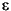) that are close to 0.

The F2XM1 instruction computes the exponential (2x - 1). This instruction only operates on source values in the range -1.0 to +1.0.

The FSCALE instruction multiplies the source operand by a power of 2.

Transcendental Instruction Accuracy

The algorithms that the Pentium and Pentium Pro processors use for the transcendental instructions (FSIN, FCOS, FSINCOS, FPTAN, FPATAN, F2XM1, FYL2X, and FYL2XP1) allow a higher level of accuracy than was possible in earlier Intel Architecture math coprocessors and FPUs. The accuracy of these instructions is measured in terms of units in the last place (ulp). For a given argument x, let f(x) and F(x) be the correct and computed (approximate) function values, respectively. The error in ulps is defined to be: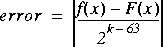where k is an integer such that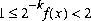.

With the Pentium and Pentium Pro processors, the worst case error on transcendental functions is less than 1 ulp when rounding to the nearest-even and less than 1.5 ulps when rounding in other modes. The functions are guaranteed to be monotonic, with respect to the input operands, throughout the domain supported by the instruction.

With the Intel486 processor and Intel 387 math coprocessor, the worst-case, transcendental-function error is typically 3 or 3.5 ulps, but is sometimes as large as 4.5 ulps.

FPU Control Instructions

The following instructions control the state and modes of operation of the FPU. They also allow the status of the FPU to be examined:

FINIT/FNINIT Initialize FPU
FSTCW/FNSTCW Store FPU control word
FSTSW/FNSTSW Store FPU status word
FCLEX/FNCLEX Clear FPU exception flags
FSTENV/FNSTENV Store FPU environment
FRSTOR Restore FPU state
FSAVE/FNSAVE Save FPU state
FINCSTP Increment FPU register stack pointer
FDECSTP Decrement FPU register stack pointer
FFREE Free FPU register
FNOP No operation
WAIT/FWAIT Check for and handle pending unmasked FPU exceptions

The FINIT/FNINIT instructions initialize the FPU and its internal registers to default values.

The FLDCW instructions loads the FPU control word register with a value from memory. The FSTCW/FNSTCW and FSTSW/FNSTSW instructions store the FPU control and status words, respectively, in memory (or for an FSTSW/FNSTSW instruction in a general-purpose register).

The FSTENV/FNSTENV and FSAVE/FNSAVE instructions save the FPU environment and state, respectively, in memory. The FPU environment includes all the FPU's control and status registers; the FPU state includes the FPU environment and the data registers in the FPU register stack. (The FSAVE/FNSAVE instruction also initializes the FPU to default values, like the FINIT/FNINIT instruction, after it saves the original state of the FPU.)

The FLDENV and FRSTOR instructions load the FPU environment and state, respectively, from memory into the FPU. These instructions are commonly used when switching tasks or contexts.

The WAIT/FWAIT instructions are synchronization instructions. (They are actually mnemonics for the same opcode.) These instructions check the FPU status word for pending unmasked FPU exceptions. If any pending unmasked FPU exceptions are found, they are handled before the processor resumes execution of the instructions (integer, floating-point, or system instruction) in the instruction stream. The WAIT/FWAIT instructions are provided to allow synchronization of instruction execution between the FPU and the processor's integer unit. See "Floating-Point Exception Synchronization" for more information on the use of the WAIT/FWAIT instructions.

Waiting Vs. Non-waiting Instructions

All of the floating-point instructions except a few special control instructions perform a wait operation (similar to the WAIT/FWAIT instructions), to check for and handle pending unmasked FPU exceptions, before they perform their primary operation (such as adding two real numbers). These instructions are called waiting instructions. Some of the FPU control instructions, such as FSTSW/FNSTSW, have both a waiting and a non-waiting versions. The waiting version (with the "F" prefix) executes a wait operation before it performs its primary operation; whereas, the non-waiting version (with the "FN" prefix) ignores pending unmasked exceptions. Non-waiting instructions allow software to save the current FPU state without first handling pending exceptions or to reset or reinitialize the FPU without regard for pending exceptions.

Note: When operating a Pentium or Intel486 processor in MS-DOS compatibility mode, it is possible (under unusual circumstances) for a non-waiting instruction to be interrupted prior to being executed to handle a pending FPU exception. The circumstances where this can happen and the resulting action of the processor are described in "No-Wait FPU Instructions Can Get FPU Interrupt in Window". When operating a Pentium Pro processor in MS-DOS compatibility mode, non-waiting instructions can not be interrupted in this way (see "MS-DOS* Compatibility Mode in the Pentium® Pro Processor").

Unsupported FPU Instructions

The Intel 8087 instructions FENI and FDISI and the Intel 287 math coprocessor instruction FSETPM perform no function in the Intel 387 math coprocessor, or the Intel486, Pentium, or Pentium Pro processors. If these opcodes are detected in the instruction stream, the FPU performs no specific operation and no internal FPU states are affected.

Operating on NaNs

As was described in "NaNs", the FPU supports two types of NaNs: SNaNs and QNaNs. An SNaN is any NaN value with its most-significant fraction bit set to 0 and at least one other fraction bit set to 1. (If all the fraction bits are set to 0, the value is an ·.) A QNaN is any NaN value with the most-significant fraction bit set to 1. The sign bit of a NaN is not interpreted.

As a general rule, when a QNaN is used in one or more arithmetic floating-point instructions, it is allowed to propagate through a computation. An SNaN on the other hand causes a floating-point invalid-operation exception to be signaled. SNaNs are typically used to trap or invoke an exception handler. They must be inserted by software; that is, the FPU never generates an SNaN as a result.

The floating-point invalid-operation exception has a flag and a mask bit associated with it in the FPU status and control registers, respectively (see "Floating-Point Exception Handling"). The mask bit determines how the FPU handles an SNaN value. If the floating-point invalid-operation mask bit is set, the SNaN is converted to a QNaN by setting the most-significant fraction bit of the value to 1. The result is then stored in the destination operand and the floating-point invalid-operation flag is set. If the invalid-operation mask is clear, a floating-point invalid-operation fault is signaled and no result is stored in the destination operand.

When a real operation or exception delivers a QNaN result, the value of the result depends on the source operands, as shown in Table 31-18.

Except for the rules given at the beginning of this section for encoding SNaNs and QNaNs, software is free to use the bits in the significand of a NaN for any purpose. Both SNaNs and QNaNs can be encoded to carry and store data, such as diagnostic information.

 Source Operands QNaN Result An SNaN and a QNaN. The QNaN source operand. Two SNaNs. The SNaN with the larger significand converted into a QNaN. Two QNaNs. The QNaN with the larger significand. An SNaN and a real value. The SNaN converted into a QNaN. A QNaN and a real value. The QNaN source operand. Neither source operand is a NaN and a floating-point invalid-operation exception is signaled. The default QNaN real indefinite.

Uses for Signaling NANs

By unmasking the invalid operation exception, the programmer can use signaling NaNs to trap to the exception handler. The generality of this approach and the large number of NaN values that are available provide the sophisticated programmer with a tool that can be applied to a variety of special situations.

For example, a compiler can use signaling NaNs as references to uninitialized (real) array elements. The compiler can preinitialize each array element with a signaling NaN whose significand contained the index (relative position) of the element. Then, if an application program attempts to access an element that it had not initialized, it can use the NaN placed there by the compiler. If the invalid operation exception is unmasked, an interrupt will occur, and the exception handler will be invoked. The exception handler can determine which element has been accessed, since the operand address field of the exception pointers will point to the NaN, and the NaN will contain the index number of the array element.

Uses for Quiet NANs

Quiet NaNs are often used to speed up debugging. In its early testing phase, a program often contains multiple errors. An exception handler can be written to save diagnostic information in memory whenever it was invoked. After storing the diagnostic data, it can supply a quiet NaN as the result of the erroneous instruction, and that NaN can point to its associated diagnostic area in memory. The program will then continue, creating a different NaN for each error. When the program ends, the NaN results can be used to access the diagnostic data saved at the time the errors occurred. Many errors can thus be diagnosed and corrected in one test run.

In embedded applications which use computed results in further computations, an undetected QNaN can invalidate all subsequent results. Such applications should therefore periodically check for QNaNs and provide a recovery mechanism to be used if a QNaN result is detected.

Floating-Point Exception Handling

The FPU detects six classes of exception conditions while executing floating-point instructions:

• Invalid operation (#I)
• Stack overflow or underflow (#IS)
• Invalid arithmetic operation (#IA)
• Divide-by-zero (#Z)
• Denormalized operand (#D)
• Numeric overflow (#O)
• Numeric underflow (#U)
• Inexact result (precision) (#P)

The nomenclature of "#" symbol followed by one or two letters (for example, #IS) is used in this manual to indicate exception conditions. It is merely a short-hand form and is not related to assembler mnemonics.

Each of the six exception classes has a corresponding flag bit in the FPU status word and a mask bit in the FPU control word (see "FPU Status Register" and "FPU Control Word", respectively). In addition, the exception summary (ES) flag in the status word indicates when any of the exceptions has been detected, and the stack fault (SF) flag (also in the status word) distinguishes between the two types of invalid-operation exceptions.

When the FPU detects a floating-point exception, it sets the appropriate flags in the FPU status word, then takes one of two possible courses of action:

• Handles the exception automatically, producing a predefined (and often times usable result), while allowing program execution to continue undisturbed.
• Invokes a software exception handler to handle the exception.

The following sections describe how the FPU handles exceptions (either automatically or by calling a software exception handler), how the FPU detects the various floating-point exceptions, and the automatic (masked) response to the floating-point exceptions.

Arithmetic vs. Non-arithmetic Instructions

When dealing with floating-point exceptions, it is useful to distinguish between arithmetic instructions and non-arithmetic instructions. Non-arithmetic instructions have no operands or do not make substantial changes to their operands. Arithmetic instructions do make significant changes to their operands; in particular, they make changes that could result in a floating-point exception being signaled. Table 31-19 lists the non-arithmetic and arithmetic instructions. It should be noted that some non-arithmetic instructions can signal a floating-point stack (fault) exception, but this exception is not the result of an operation on an operand.

Automatic Exception Handling

If the FPU detects an exception condition for a masked exception (an exception with its mask bit set), it sets the exception flag for the exception and delivers a predefined (default) response and continues executing instructions. The masked (default) responses to exceptions have been chosen to deliver a reasonable result for each exception condition and are generally satisfactory for most floating-point applications. By masking or unmasking specific floating-point exceptions in the FPU control word, programmers can delegate responsibility for most exceptions to the FPU and reserve the most severe exception conditions for software exception handlers.

Because the exception flags are "sticky," they provide a cumulative record of the exceptions that have occurred since they were last cleared. A programmer can thus mask all exceptions, run a calculation, and then inspect the exception flags to see if any exceptions were detected during the calculation.

Arithmetic and Non-arithmetic Instructions (Sheet 1 of 2)
Non-arithmetic Instructions   Arithmetic Instructions
FABS   F2XM1
FCLEX   FBLD
FDECSTP   FBSTP
FFREE   FCOM/FCOMP/FCOMPP
FINCSTP   FCOS
FINIT/FNINIT   FDIV/FDIVP/FDIVR/FDIVRP
FLD (extended format from memory)   FICOM/FICOMP
FLD constant   FIDIV/FIDIVR
FLDCW   FILD
FLDENV   FIMUL
FNOP   FIST/FISTP
FRSTOR   FISUB/FISUBR
FSAVE/FNSAVE   FLD (conversion)
FST/FSTP (register-to-register)   FMUL/FMULP
FSTP (extended format to memory)   FPATAN
FSTCW/FNSTCW   FPREM/FPREM1
FSTENV/FNSTENV   FPTAN
FSTSW/FNSTSW   FRNDINT
WAIT/FWAIT   FSCALE
FXAM   FSIN
FXCH   FSINCOS
FSQRT
FST/FSTP (conversion)
FSUB/FSUBP/FSUBR/FSUBRP
FTST
FUCOM/FUCOMP/FUCOMPP
FXTRACT
FYL2X/FYL2XP1

Note that when exceptions are masked, the FPU may detect multiple exceptions in a single instruction, because it continues executing the instruction after performing its masked response. For example, the FPU can detect a denormalized operand, perform its masked response to this exception, and then detect numeric underflow.

Software Exception Handling

The FPU in the Pentium Pro, Pentium, and Intel486 processors provides two different modes of operation for invoking a software exception handler for floating-point exceptions: native mode and MS-DOS compatibility mode. The mode of operation is selected with the NE flag of control register CR0. (See Chapter 2, System Architecture Overview, in the Intel Architecture Software Developer's Manual, Volume 3, for more information about the NE flag.)

Native Mode

The native mode for handling floating-point exceptions is selected by setting the NE flag in control register CR0 to 1. In this mode, if the FPU detects an exception condition while executing a floating-point instruction and the exception is unmasked (the mask bit for the exception is cleared), the FPU sets the flag for the exception and the ES flag in the FPU status word. It then invokes the software exception handler through the floating-point-error exception (#MF, vector 16), immediately before execution of any of the following instructions in the processor's instruction stream:

• The next floating-point instruction, unless it is one of the non-waiting instructions (FNINIT, FNCLEX, FNSTSW, FNSTCW, FNSTENV, and FNSAVE).
• The next WAIT/FWAIT instruction.
• The next MMX instruction.

If the next floating-point instruction in the instruction stream is a non-waiting instruction, the FPU executes the instruction without invoking the software exception handler.

MS-DOS* Compatibility Mode

If the NE flag in control register CR0 is set to 0, the MS-DOS compatibility mode for handling floating-point exceptions is selected. In this mode, the software exception handler for floating-point exceptions is invoked externally using the processor's FERR#, INTR, and IGNNE# pins. This method of reporting floating-point errors and invoking an exception handler is provided to support the floating-point exception handling mechanism used in PC systems that are running the MS-DOS or Windows* 95 operating system.

The MS-DOS compatibility mode is typically used as follows to invoke the floating-point exception handler:

1. If the FPU detects an unmasked floating-point exception, it sets the flag for the exception and the ES flag in the FPU status word.

2. If the IGNNE# pin is deasserted, the FPU then asserts the FERR# pin either immediately, or else delayed (deferred) until just before the execution of the next waiting floating-point instruction or MMX™ instruction. Whether the FERR# pin is asserted immediately or delayed depends on the type of processor, the instruction, and the type of exception.

3. If a preceding floating-point instruction has set the exception flag for an unmasked FPU exception, the processor freezes just before executing the next WAIT instruction, waiting floating-point instruction, or MMX instruction. Whether the FERR# pin was asserted at the preceding floating-point instruction or is just now being asserted, the freezing of the processor assures that the FPU exception handler will be invoked before the new floatingpoint (or MMX) instruction gets executed.

4. The FERR# pin is connected through external hardware to IRQ13 of a cascaded, programmable interrupt controller (PIC). When the FERR# pin is asserted, the PIC is programmed to generate an interrupt 75H.

5. The PIC asserts the INTR pin on the processor to signal the interrupt 75H.

6. The BIOS for the PC system handles the interrupt 75H by branching to the interrupt 2 (NMI) interrupt handler.

7. The interrupt 2 handler determines if the interrupt is the result of an NMI interrupt or a floating-point exception.

8. If a floating-point exception is detected, the interrupt 2 handler branches to the floating-point exception handler.

If the IGNNE# pin is asserted, the processor ignores floating-point error conditions. This pin is provided to inhibit floating-point exceptions from being generated while the floating-point exception handler is servicing a previously signaled floating-point exception.

"Guidelines for Writing FPU Exception Handlers", describes the MS-DOS compatibility mode in much greater detail.

Typical Floating-Point Exception Handler Actions

After the floating-point exception handler is invoked, the processor handles the exception in the same manner that it handles non-FPU exceptions. (The floating-point exception handler is normally part of the operating system or executive software.) A typical action of the exception handler is to store FPU state information in memory (with the FSTENV/FNSTENV or FSAVE/FNSAVE instructions) so that it can evaluate the exception and formulate an appropriate response (see "Saving the FPU's State"). Other typical exception handler actions include:

• Examining stored FPU state information (control, status, and tag words, and FPU instruction and operand pointers) to determine the nature of the error.
• Correcting the condition that caused the error.
• Clearing the exception bits in the status word.
• Returning to the interrupted program and resuming normal execution.

If the faulting floating-point instruction is followed by one or more non-floating-point instructions, it may not be useful to re-execute the faulting instruction. See "Floating-Point Exception Synchronization", for more information on synchronizing floating-point exceptions.

In cases where the handler needs to restart program execution with the faulting instruction, the IRET instruction cannot be used directly. The reason for this is that because the exception is not generated until the next floating-point or WAIT/FWAIT instruction following the faulting floating-point instruction, the return instruction pointer on the stack may not point to the faulting instruction. To restart program execution at the faulting instruction, the exception handler must obtain a pointer to the instruction from the saved FPU state information, load it into the return instruction pointer location on the stack, and then execute the IRET instruction.

In lieu of writing recovery procedures, the exception handler can do the following:

• Increment an exception counter for later display or printing.
• Print or display diagnostic information (such as, the FPU environment and registers).
• Halt further program execution.

See "FPU Exception Handling Examples", for general examples of floating-point exception handlers and for specific examples of how to write a floating-point exception handler when using the MS-DOS compatibility mode.

Floating-Point Exception Conditions

The following sections describe the various conditions that cause a floating-point exception to be generated and the masked response of the FPU when these conditions are detected. Chapter 3, Instruction Set Reference, in the Intel Architecture Software Developer's Manual, Volume 2, lists the floating-point exceptions that can be signaled for each floating-point instruction.

Invalid Operation Exception

The floating-point invalid-operation exception occurs in response to two general types of operations:

• Stack overflow or underflow (#IS).
• Invalid arithmetic operand (#IA).

The flag for this exception (IE) is bit 0 of the FPU status word, and the mask bit (IM) is bit 0 of the FPU control word. The stack fault flag (SF) of the FPU status word indicates the type of operation caused the exception. When the SF flag is set to 1, a stack operation has resulted in stack overflow or underflow; when the flag is cleared to 0, an arithmetic instruction has encountered an invalid operand. Note that the FPU explicitly sets the SF flag when it detects a stack overflow or underflow condition, but it does not explicitly clear the flag when it detects an invalid-arithmetic-operand condition. As a result, the state of the SF flag can be 1 following an invalid-arithmetic-operation exception, if it was not cleared from the last time a stack overflow or underflow condition occurred. See "Stack Fault Flag", for more information about the SF flag.

Stack Overflow or Underflow Exception (#IS)

The FPU tag word keeps track of the contents of the registers in the FPU register stack (see "FPU Tag Word"). It then uses this information to detect two different types of stack faults:

• Stack overflow-an instruction attempts to write a value into a non-empty FPU register
• Stack underflow-an instruction attempts to read a value from an empty FPU register.

When the FPU detects stack overflow or underflow, it sets the IE flag (bit 0) and the SF flag (bit 6) in the FPU status word to 1. It then sets condition-code flag C1 (bit 9) in the FPU status word to 1 if stack overflow occurred or to 0 if stack underflow occurred.

If the invalid-operation exception is masked, the FPU then returns the real, integer, or BCD-integer indefinite value to the destination operand, depending on the instruction being executed. This value overwrites the destination register or memory location specified by the instruction.

If the invalid-operation exception is not masked, a software exception handler is invoked (see "Software Exception Handling") and the top-of-stack pointer (TOP) and source operands remain unchanged.

The term stack overflow comes from the condition where the a program has pushed eight values onto the FPU register stack and the next value pushed on the stack causes a stack wraparound to a register that already contains a value. The term stack underflow refers to the opposite condition from stack overflow. Here, a program has popped eight values from the FPU register stack and the next value popped from the stack causes stack wraparound to an empty register.

Invalid Arithmetic Operand Exception (#IA)

The FPU is able to detect a variety of invalid arithmetic operations that can be coded in a program. These operations generally indicate a programming error, such as dividing · by ·. Table 31-20 lists the invalid arithmetic operations that the FPU detects. This group includes the invalid operations defined in IEEE Std. 854.

When the FPU detects an invalid arithmetic operand, it sets the IE flag (bit 0) in the FPU status word to 1. If the invalid-operation exception is masked, the FPU then returns an indefinite value to the destination operand or sets the floating-point condition codes, as shown in Table 31-20. If the invalid-operation exception is not masked, a software exception handler is invoked (see "Software Exception Handling") and the top-of-stack pointer (TOP) and source operands remain unchanged.

 Condition Masked Response Any arithmetic operation on an operand that is in an unsupported format. Return the real indefinite value to the destination operand. Any arithmetic operation on a SNaN. Return a QNaN to the destination operand (see "Operating on NaNs"). Compare and test operations: one or both operands are NaNs. Set the condition code flags (C0, C2, and C3) in the FPU status word to 111B (not comparable). Addition: operands are opposite-signed infinities.Subtraction: operands are like-signed infinities. Return the real indefinite value to the destination operand. Multiplication: · by 0; 0 by ·. Return the real indefinite value to the destination operand. Division: · by ·; 0 by 0. Return the real indefinite value to the destination operand. Remainder instructions FPREM, FPREM1: modulus (divisor) is 0 or dividend is ·. Return the real indefinite; clear condition code flag C2 to 0. Trigonometric instructions FCOS, FPTAN, FSIN, FSINCOS: source operand is ·. Return the real indefinite; clear condition code flag C2 to 0. FSQRT: negative operand (except FSQRT (-0) = -0); FYL2X: negative operand (except FYL2X (-0) = -·); FYL2XP1: operand more negative than -1. Return the real indefinite value to the destination operand. FBSTP: source register is empty or it contains a NaN, ·, or a value that cannot be represented in 18 decimal digits. Store BCD integer indefinite value in the destination operand. FXCH: one or both registers are tagged empty. Load empty registers with the real indefinite value, then perform the exchange. FIST/FISTP instruction when input operand <> MAXINT for destination operand size. Return MAXNEG to destination operand.

Divide-By-Zero Exception (#Z)

The FPU reports a floating-point zero-divide exception whenever an instruction attempts to divide a finite non-zero operand by 0. The flag (ZE) for this exception is bit 2 of the FPU status word, and the mask bit (ZM) is bit 2 of the FPU control word. The FDIV, FDIVP, FDIVR, FDIVRP, FIDIV, and FIDIVR instructions and the other instructions that perform division internally (FYL2X and FXTRACT) can report the divide-by-zero exception.

When a divide-by-zero exception occurs and the exception is masked, the FPU sets the ZE flag and returns the values shown in Table 7-21. If the divide-by-zero exception is not masked, the ZE flag is set, a software exception handler is invoked (see "Software Exception Handling"), and the top-of-stack pointer (TOP) and source operands remain unchanged.

 Condition Masked Response Divide or reverse divide operation with a 0 divisor. Returns an · signed with the exclusive OR of the sign of the two operands to the destination operand. FYL2X instruction. Returns an · signed with the opposite sign of the non-zero operand to the destination operand. FXTRACT instruction. ST(1) is set to -·; ST(0) is set to 0 with the same sign as the source operand.

Denormal Operand Exception (#D)

The FPU signals the denormal-operand exception under the following conditions:

• If an arithmetic instruction attempts to operate on a denormal operand (see "Normalized and Denormalized Finite Numbers").
• If an attempt is made to load a denormal single- or double-real value into an FPU register. (If the denormal value being loaded is an extended-real value, the denormal-operand exception is not reported.)

The flag (DE) for this exception is bit 1 of the FPU status word, and the mask bit (DM) is bit 1 of the FPU control word.

When a denormal-operand exception occurs and the exception is masked, the FPU sets the DE flag, then proceeds with the instruction. The denormal operand in single- or double-real format is automatically normalized when converted to the extended-real format. Operating on denormal numbers will produce results at least as good as, and often better than, what can be obtained when denormal numbers are flushed to zero. In fact, subsequent operations will benefit from the additional precision of the internal extended-real format. Most programmers mask this exception so that a computation may proceed, then analyze any loss of accuracy when the final result is delivered.

When a denormal-operand exception occurs and the exception is not masked, the DE flag is set and a software exception handler is invoked (see "Software Exception Handling"). The top-of-stack pointer (TOP) and source operands remain unchanged. When denormal operands have reduced significance due to loss of low-order bits, it may be advisable to not operate on them. Precluding denormal operands from computations can be accomplished by an exception handler that responds to unmasked denormal-operand exceptions.

Numeric Overflow Exception (#O)

The FPU reports a floating-point numeric overflow exception (#O) whenever the rounded result of an arithmetic instruction exceeds the largest allowable finite value that will fit into the real format of the destination operand. For example, if the destination format is extended-real (80 bits), overflow occurs when the rounded result falls outside the unbiased range of -1.0 * 216384 to 1.0 * 216384 (exclusive). Numeric overflow can occur on arithmetic operations where the result is stored in an FPU data register. It can also occur on store-real operations (with the FST and FSTP instructions), where a within-range value in a data register is stored in memory in a single- or double-real format. The overflow threshold range for the single-real format is -1.0 * 2128 to 1.0 * 2128; the range for the double-real format is -1.0 * 21024 to 1.0 * 21024.

The numeric overflow exception cannot occur when overflow occurs when storing values in an integer or BCD integer format. Instead, the invalid-arithmetic-operand exception is signaled.

The flag (OE) for the numeric-overflow exception is bit 3 of the FPU status word, and the mask bit (OM) is bit 3 of the FPU control word.

When a numeric-overflow exception occurs and the exception is masked, the FPU sets the OE flag and returns one of the values shown in Table 31-22. The value returned depends on the current rounding mode of the FPU (see "Rounding Control Field").

.
 Rounding Mode Sign of True Result Result To nearest + +· - -· Toward -· + Largest finite positive number - -· Toward +· + +· - Largest finite negative number Toward zero + Largest finite positive number - Largest finite negative number

The action that the FPU takes when numeric overflow occurs and the numeric-overflow exception is not masked, depends on whether the instruction is supposed to store the result in memory or on the register stack.

If the destination is a memory location, the OE flag is set and a software exception handler is invoked (see "Software Exception Handling"). The top-of-stack pointer (TOP) and source and destination operands remain unchanged.

If the destination is the register stack, the exponent of the rounded result is divided by 224576 and the result is stored along with the significand in the destination operand. Condition code bit C1 in the FPU status word (called in this situation the "round-up bit") is set if the significand was rounded upward and cleared if the result was rounded toward 0. After the result is stored, the OE flag is set and a software exception handler is invoked.

The scaling bias value 24,576 is equal to 3 * 213. Biasing the exponent by 24,576 normally translates the number as nearly as possible to the middle of the extended-real exponent range so that, if desired, it can be used in subsequent scaled operations with less risk of causing further exceptions.

When using the FSCALE instruction, massive overflow can occur, where the result is too large to be represented, even with a bias-adjusted exponent. Here, if overflow occurs again, after the result has been biased, a properly signed · is stored in the destination operand.

Numeric Underflow Exception (#U)

The FPU reports a floating-point numeric underflow exception (#U) whenever the rounded result of an arithmetic instruction is "tiny" (that is, less than the smallest possible normalized, finite value that will fit into the real format of the destination operand). For example, if the destination format is extended-real (80 bits), underflow occurs when the rounded result falls in the unbiased range of -1.0 * 2-16382 to 1.0 * 2-16382 (exclusive). Like numeric overflow, numeric underflow can occur on arithmetic operations where the result is stored in an FPU data register. It can also occur on store-real operations (with the FST and FSTP instructions), where a within-range value in a data register is stored in memory in a single- or double-real format. The underflow threshold range for the single-real format is -1.0 * 2-126 to 1.0 * 2-126; the range for the double-real format is -1.0 * 2-1022 to 1.0 * 2-1022. (The numeric underflow exception cannot occur when storing values in an integer or BCD integer format.)

The flag (UE) for the numeric-underflow exception is bit 4 of the FPU status word, and the mask bit (UM) is bit 4 of the FPU control word.

When a numeric-underflow exception occurs and the exception is masked, the FPU denormalizes the result (see "Normalized and Denormalized Finite Numbers"). If the denormalized result is exact, the FPU stores the result in the destination operand, without setting the UE flag. If the denormal result is inexact, the FPU sets the UE flag, then goes on to handle the inexact-result exception condition (see "Inexact-Result (Precision) Exception (#P)"). It is important to note that if numeric-underflow is masked, a numeric-underflow exception is signaled only if the denormalized result is inexact. If the denormalized result is exact, no flags are set and no exceptions are signaled.

The action that the FPU takes when numeric underflow occurs and the numeric-underflow exception is not masked, depends on whether the instruction is supposed to store the result in memory or on the register stack.

If the destination is a memory location, the UE flag is set and a software exception handler is invoked (see "Software Exception Handling"). The top-of-stack pointer (TOP) and source and destination operands remain unchanged.

If the destination is the register stack, the exponent of the rounded result is multiplied by 224576 and the product is stored along with the significand in the destination operand. Condition code bit C1 in the FPU the status register (acting here as a "round-up bit") is set if the significand was rounded upward and cleared if the result was rounded toward 0. After the result is stored, the UE flag is set and a software exception handler is invoked.

The scaling bias value 24,576 is the same as is used for the overflow exception and has the same effect, which is to translate the result as nearly as possible to the middle of the extended-real exponent range.

When using the FSCALE instruction, massive underflow can occur, where the result is too tiny to be represented, even with a bias-adjusted exponent. Here, if underflow occurs again, after the result has been biased, a properly signed 0 is stored in the destination operand.

Inexact-Result (Precision) Exception (#P)

The inexact-result exception (also called the precision exception) occurs if the result of an operation is not exactly representable in the destination format. For example, the fraction 1/3 cannot be precisely represented in binary form. This exception occurs frequently and indicates that some (normally acceptable) accuracy has been lost. The exception is supported for applications that need to perform exact arithmetic only. Because the rounded result is generally satisfactory for most applications, this exception is commonly masked. Note that the transcendental instructions [FSIN, FCOS, FSINCOS, FPTAN, FPATAN, F2XM1, FYL2X, and FYL2XP1] by nature produce inexact results.

The inexact-result exception flag (PE) is bit 5 of the FPU status word, and the mask bit (PM) is bit 5 of the FPU control word.

If the inexact-result exception is masked when an inexact-result condition occurs and a numeric overflow or underflow condition has not occurred, the FPU sets the PE flag and stores the rounded result in the destination operand. The current rounding mode determines the method used to round the result (see "Rounding Control Field"). The C1 (round-up) bit in the FPU status word indicates whether the inexact result was rounded up (C1 is set) or "not rounded up" (C1 is cleared). In the "not rounded up" case, the least-significant bits of the inexact result are truncated so that the result fits in the destination format.

If the inexact-result exception is not masked when an inexact result occurs and numeric overflow or underflow has not occurred, the FPU performs the same operation described in the previous paragraph and, in addition, invokes a software exception handler (see "Software Exception Handling").

If an inexact result occurs in conjunction with numeric overflow or underflow, one of the following operations is carried out:

• If an inexact result occurs along with masked overflow or underflow, the OE or UE flag and the PE flag are set and the result is stored as described for the overflow or underflow exceptions (see "Numeric Overflow Exception (#O)" or "Numeric Underflow Exception (#U)"). If the inexact-result exception is unmasked, the FPU also invokes the software exception handler.
• If an inexact result occurs along with unmasked overflow or underflow and the destination operand is a register, the OE or UE flag and the PE flag are set, the result is stored as described for the overflow or underflow exceptions, and the software exception handler is invoked.
• If an inexact result occurs along with unmasked overflow or underflow and the destination operand is a memory location, the inexact-result condition is ignored.

Exception Priority

The processor handles exceptions according to a predetermined precedence. When an instruction generates two or more exception conditions, the exception precedence sometimes results in the higher-priority exception being handled and the lower-priority exceptions being ignored. For example, dividing an SNaN by zero can potentially signal an invalid-arithmetic-operand exception (due to the SNaN operand) and a divide-by-zero exception. Here, if both exceptions are masked, the FPU handles the higher-priority exception only (the invalid-arithmetic-operand exception), returning a real indefinite to the destination. Alternately, a denormal-operand or inexact-result exception can accompany a numeric underflow or overflow exception, with both exceptions being handled.

The precedence for floating-point exceptions is as follows:

1. Invalid-operation exception, subdivided as follows:

a. Stack underflow.

b. Stack overflow.

c. Operand of unsupported format.

d. SNaN operand.

2. QNaN operand. Though this is not an exception, the handling of a QNaN operand has precedence over lower-priority exceptions. For example, a QNaN divided by zero results in a QNaN, not a zero-divide exception.

3. Any other invalid-operation exception not mentioned above or a divide-by-zero exception.

4. Denormal-operand exception. If masked, then instruction execution continues, and a lower-priority exception can occur as well.

5. Numeric overflow and underflow exceptions in conjunction with the inexact-result exception.

6. Inexact-result exception.

Invalid operation, zero divide, and denormal operand exceptions are detected before a floating-point operation begins, whereas overflow, underflow, and precision errors are not detected until a true result has been computed. When a pre-operation exception is detected, the FPU register stack and memory have not yet been updated, and appear as if the offending instructions has not been executed. When a post-operation exception is detected, the register stack and memory may be updated with a result (depending on the nature of the error).

Floating-Point Exception Synchronization

Because the integer unit and FPU are separate execution units, it is possible for the processor to execute floating-point, integer, and system instructions concurrently. No special programming techniques are required to gain the advantages of concurrent execution. (Floating-point instructions are placed in the instruction stream along with the integer and system instructions.) However, concurrent execution can cause problems for floating-point exception handlers.

This problem is related to the way the FPU signals the existence of unmasked floating-point exceptions. (Special exception synchronization is not required for masked floating-point exceptions, because the FPU always returns a masked result to the destination operand.)

When a floating-point exception is unmasked and the exception condition occurs, the FPU stops further execution of the floating-point instruction and signals the exception event. On the next occurrence of a floating-point instruction or a WAIT/FWAIT instruction in the instruction stream, the processor checks the ES flag in the FPU status word for pending floating-point exceptions. It floating-point exceptions are pending, the FPU makes an implicit call (traps) to the floating-point software exception handler. The exception handler can then execute recovery procedures for selected or all floating-point exceptions.

Synchronization problems occur in the time frame between when the exception is signaled and when it is actually handled. Because of concurrent execution, integer or system instructions can be executed during this time frame. It is thus possible for the source or destination operands for a floating-point instruction that faulted to be overwritten in memory, making it impossible for the exception handler to analyze or recover from the exception.

To solve this problem, an exception synchronizing instruction (either a floating-point instruction or a WAIT/FWAIT instruction) can be placed immediately after any floating-point instruction that might present a situation where state information pertaining to a floating-point exception might be lost or corrupted. Floating-point instructions that store data in memory are prime candidates for synchronization. For example, the following three lines of code have the potential for exception synchronization problems:

```FILD COUNT ; Floating-point instructionINC COUNT ; Integer instructionFSQRT ; Subsequent floating-point instruction```

In this example, the INC instruction modifies the result of a floating-point instruction (FILD). If an exception is signaled during the execution of the FILD instruction, the result stored in the COUNT memory location might be overwritten before the exception handler is called.

Rearranging the instructions, as follows, so that the FSQRT instruction follows the FILD instruction, synchronizes the exception handling and eliminates the possibility of the exception being handled incorrectly.

```FILD COUNT ; Floating-point instructionFSQRT ; Subsequent floating-point instruction synchronizes; any exceptions generated by the FILD instruction.INC COUNT ; Integer instruction```

The FSQRT instruction does not require any synchronization, because the results of this instruction are stored in the FPU data registers and will remain there, undisturbed, until the next floating-point or WAIT/FWAIT instruction is executed. To absolutely insure that any exceptions emanating from the FSQRT instruction are handled (for example, prior to a procedure call), a WAIT instruction can be placed directly after the FSQRT instruction.

Note that some floating-point instructions (non-waiting instructions) do not check for pending unmasked exceptions (see "FPU Control Instructions"). They include the FNINIT, FNSTENV, FNSAVE, FNSTSW, FNSTCW, and FNCLEX instructions. When an FNINIT, FNSTENV, FNSAVE, or FNCLEX instruction is executed, all pending exceptions are essentially lost (either the FPU status register is cleared or all exceptions are masked). The FNSTSW and FNSTCW instructions do not check for pending interrupts, but they do not modify the FPU status and control registers. A subsequent "waiting" floating-point instruction can then handle any pending exceptions.

Floating-Point Exceptions Summary

Table 31-23 lists the floating-point instruction mnemonics in alphabetical order. For each mnemonic, it summarizes the exceptions that the instruction may cause. See "Floating-Point Exception Conditions", for a detailed discussion of the floating-point exceptions. The following codes indicate the floating-point exceptions:

#IS

Invalid-operation exception for stack underflow or stack overflow.

#IA

Invalid-operation exception for invalid arithmetic operands and
unsupported formats.

#D

Denormal-operand exception.

#Z

Divide-by-zero exception.

#O

Numeric-overflow exception.

#U

Numeric-underflow exception.

#P

Inexact-result (precision) exception.

 Mnemonic Instruction #IS #IA #D #Z #O #U #P F2XM1 2X-1 Y Y Y Y Y FABS Absolute value Y FADD(P) Add real Y Y Y Y Y Y FBLD BCD load Y FBSTP BCD store and pop Y Y Y FCHS Change sign Y FCLEX Clear exceptions FCMOVcc Floating-point conditional move Y FCOM, FCOMP, FCOMPP Compare real Y Y Y FCOMI, FCOMIP, FUCOMI, FUCOMIP Compare real and set EFLAGS Y Y FCOS Cosine Y Y Y Y Y FDECSTP Decrement stack pointer FDIV(R)(P) Divide real Y Y Y Y Y Y Y FFREE Free register Mnemonic Instruction #IS #IA #D #Z #O #U #P FIADD Integer add Y Y Y Y Y Y FICOM(P) Integer compare Y Y Y FIDIV Integer divide Y Y Y Y Y Y FIDIVR Integer divide reversed Y Y Y Y Y Y Y FILD Integer load Y FIMUL Integer multiply Y Y Y Y Y Y FINCSTP Increment stack pointer FINIT Initialize processor FIST(P) Integer store Y Y Y FISUB(R) Integer subtract Y Y Y Y Y Y FLD extended or stack Load real Y FLD single or double Load real Y Y Y FLD1 Load + 1.0 Y FLDCW Load Control word Y Y Y Y Y Y Y FLDENV Load environment Y Y Y Y Y Y Y FLDL2E Load log2e Y FLDL2T Load log210 Y FLDLG2 Load log102 Y FLDLN2 Load loge2 Y FLDPI LoadY FLDZ Load + 0.0 Y FMUL(P) Multiply real Y Y Y Y Y Y FNOP No operation FPATAN Partial arctangent Y Y Y Y Y FPREM Partial remainder Y Y Y Y FPREM1 IEEE partial remainder Y Y Y Y FPTAN Partial tangent Y Y Y Y Y FRNDINT Round to integer Y Y Y Y FRSTOR Restore state Y Y Y Y Y Y Y FSAVE Save state FSCALE Scale Y Y Y Y Y Y FSIN Sine Y Y Y Y Y FSINCOS Sine and cosine Y Y Y Y Y Mnemonic Instruction #IS #IA #D #Z #O #U #P FSQRT Square root Y Y Y Y FST(P) stack or extended Store real Y FST(P) single or double Store real Y Y Y Y Y Y FSTCW Store control word FSTENV Store environment FSTSW (AX) Store status word FSUB(R)(P) Subtract real Y Y Y Y Y Y FTST Test Y Y Y FUCOM(P)(P) Unordered compare real Y Y Y FWAIT CPU Wait FXAM Examine FXCH Exchange registers Y FXTRACT Extract Y Y Y Y FYL2X Y · log2X Y Y Y Y Y Y Y FYL2XP1 Y · log2(X + 1) Y Y Y Y Y

saved from Pentium® Processor - Floating-Point Unit, created 10 May 1999.

This document is also available in PDF format.